2016-05-06 21:54:04 qq_20602929 阅读数 6011
• ###### 多角度带你编写更规范的黑盒测试用例

讲解方式 通过类比生活中的例子，轻松理解测试用例的设计方法。课程内容条理清晰，目标明确，由浅入深，环环相扣。重点部分进行额外梳理和总结，更易理解和吸收。 课程亮点 1，测试用例的要素讲解，让测试用例的编写更加规范 2、多种测试用例方法的学习，让用例设计更加全面。测试用例的设计方法：等价类、边界值、判定表、因果图、状态迁移图、场景法、正交实验法、错误推测法 3，多角度的案例实践，以理解各种方法的应用 课程内容 1、测试用例的定义和组成要素 2、测试用例的设计方法：等价类、边界值、判定表、因果图、状态迁移图、场景法、正交实验法、错误推测法 3：每种设计方法对应的案例讲解 适用人群 1、对软件测试感兴趣的在校生及应届生。 2、希望转行软件测试的在职人员。 3、希望巩固软件测试设计方法的测试同行。 4、对软件测试感兴趣的其他听众。

626 人正在学习 去看看 传智

# 离散傅里叶变换

## 一维离散傅里叶变换

F(u)=N1x=0f(x)ej2πuxN$F(u)=\sum_{x=0}^{N-1} f(x) e^{-j \frac{2\pi u x}{N}}$

f(x)=1NN1x=0F(u)ej2πuxN$f(x)=\frac1N \sum_{x=0}^{N-1} F(u)e^{j \frac{2\pi u x}{N}}$

f(x)和F(u)为离散傅里叶变换对，表示为：f(x)F(u)$f(x)\leftrightarrows F(u)$
W=ej2πN$W=e^{-j\frac{2\pi}{N}}$,则一维的DFT和IDFT表示为：
F(u)=N1x=0f(x)Wux$F(u)=\sum_{x=0}^{N-1} f(x) W^{ux}$
f(x)=1NN1x=0F(u)Wux$f(x)=\frac1N \sum_{x=0}^{N-1} F(u)W^{-ux}$

## 一维快速傅里叶变换

FFT原理

Wu±rN=Wu$W^{u\pm rN}=W^{u}$
Wu±N2=Wu$W^{u\pm \frac N2}=-W^u$FFT算法推导
W因子如下特性:
Wk2N=Wk2N$W_{2N}^k =W_N^{\frac k2}$
DFT可以表示为：
F(u)=N/21x=0f(2x)W2uxN+N/21x=0f(2x+1)Wu(2x+1)N=N/21x=0f(2x)WuxN/2+N/21x=0f(2x+1)WuxN/2WuN$F(u)=\sum_{x=0}^{N/2-1} f(2x)W_N^{2ux}+\sum_{x=0}^{N/2-1}f(2x+1)W_N^{u(2x+1)}=\sum_{x=0}^{N/2-1}f(2x) W_{N/2}^{ux}+\sum_{x=0}^{N/2-1} f(2x+1)W_{N/2}^{ux}W_N^{u}$

F(u)=Fe(u)+WuNF0(u)$F(u)=F_e(u)+W_N^u F_0(u)$

F(u+M)=Fe(u)WuNF0(u)$F(u+M)=F_e(u)-W_N^u F_0(u)$## 二维离散傅里叶变换I=imread('106.jpg');
subplot(1,3,1),imshow(I),title('106 原图');
grayI=rgb2gray(I);
%彩色图像灰度化
DFTI=fftshift(fft2(grayI));
%计算傅里叶变换并移位
%求模
%求模的最大值
%求模的最小值
%将模规格化到[0 100]
subplot(1,3,3),imshow(ADFTI),title('106 频谱图2');## 二维离散傅里叶变换的性质

1）可分性
F(u,v)=M1x=0N1y=0f(x,y)ej2πxuMej2πyuN=Fx{Fy[f(x,y)]}$F(u,v)=\sum_{x=0}^{M-1}\sum_{y=0}^{N-1}f(x,y) \cdot e^{-j2\pi \frac{xu}{M}} \cdot e^{-j2\pi \frac{yu}{N}}=F_x\{ F_y[f(x,y)]\}$2）线性
F[a1f1(x,y)+a2f2(x,y)]=a1F[f1(x,y)]+a2F[f2(x,y)]$F[a_1 f_1(x,y)+a_2f_2(x,y)]=a_1F[f_1(x,y)]+a_2F[f_2(x,y)]$

3）共轭对称性
f(x,y)F(u,v)F(u,v)f(x,y)DFT$f(x,y)\leftrightarrows F(u,v)，F^*(-u,-v)是f(-x,-y)的DFT共轭函数，则$
F(u,v)=F(u,v)$F(u,v)=F^*(-u,-v)$

4）平移性

5）旋转性

f(γ,θ+θ0)F(k,ϕ+θ0)$f(\gamma,\theta+\theta_0)\leftrightarrows F(k,\phi+\theta_0)$

6）比例的变换特性
f(ax,bx)1|ab|F(ua,vb)$f(ax,bx)\leftrightarrows \frac{1}{|ab|}F(\frac ua,\frac vb)$

7）帕斯瓦尔定理

M1x=0N1y=0|f(x,y)|2=M1u=0N1v=0|F(u,v)|2$\sum_{x=0}^{M-1} \sum_{y=0}^{N-1} |f(x,y)|^2 =\sum_{u=0}^{M-1} \sum_{v=0}^{N-1} |F(u,v)|^2$

8）卷积定理

f(x,y)g(x,y)F(u,v)G(u,v)$f(x,y)*g(x,y)\leftrightarrows F(u,v)\cdot G(u,v)$
f(x,y)g(x,y)F(u,v)G(u,v)$f(x,y)\cdot g(x,y)\leftrightarrows F(u,v)* G(u,v)$

## 傅里叶变换在图像处理中的应用

1）傅里叶描绘子

2）图像滤波中应用

3）图像压缩中应用

4）卷积特性

fg=gf$f_g=g*f$
Fg(u,v)=G(u,v)F(u,v)$F_g(u,v)=G(u,v)\cdot F(u,v)$
fg=IDFT(Fg)$f_g={IDFT}(F_g)$
，先分别逆变换后点乘再逆变换得到f，降低卷积计算量

# 离散余弦变换

## 一维离散余弦变换

DCT:
F(u)=C(u)2NN1x=0f(x)cos(2x+1)uπ2N$F(u)=C(u)\sqrt{\frac 2N} \sum_{x=0}^{N-1}f(x) \cos \dfrac{(2x+1)u\pi}{2N}$
u=0,...,N1$u=0,...,N-1$

IDCT:
f(x)=2NN1x=0C(u)F(u)cos(2x+1)uπ2N$f(x)=\sqrt{\frac 2N} \sum_{x=0}^{N-1}C(u) F(u) \cos \dfrac{(2x+1)u\pi}{2N}$
x=0,...,N1$x=0,...,N-1$

C(u)={ 121u=0u=1,...,N1 $C(u) = \left\{\begin{array}{rcl}\ \frac{1}{\sqrt 2}&&u=0\\1 && u=1,...,N-1\ \end{array}\right.$## 二维离散余弦变换

DCT:
F(u,v)=2MNC(u)C(v)M1x=0N1y=0f(x,y)cos(2x+1)uπ2Ncos(2y+1)vπ2M$F(u,v)=\dfrac{2}{\sqrt{MN}}C(u)C(v) \sum_{x=0}^{M-1} \sum_{y=0}^{N-1}f(x,y) \cos \dfrac{(2x+1)u\pi}{2N} \cos \dfrac{(2y+1)v\pi}{2M}$
x,u=0,...,M1$x,u=0,...,M-1$

IDCT:
f(x,y)=2MNM1u=0N1v=0C(u)C(v)F(u,v)cos(2x+1)uπ2Ncos(2y+1)vπ2M$f(x,y)=\dfrac{2}{\sqrt{MN}}\sum_{u=0}^{M-1} \sum_{v=0}^{N-1}C(u) C(v)F(u,v) \cos \dfrac{(2x+1)u\pi}{2N} \cos \dfrac{(2y+1)v\pi}{2M}$
y,v=0,...,N1$y,v=0,...,N-1$

C(u),C(v)={ 121u,v=0u,v=1,...,N1 $C(u) ,C(v)= \left\{\begin{array}{rcl}\ \frac{1}{\sqrt 2}&&u,v=0\\1 && u,v=1,...,N-1\ \end{array}\right.$I=imread('099.jpg');
figure;
imshow(I),title(' 原图');
grayI=rgb2gray(I);
DCTI=dct2(grayI);
%离散余弦变换
%将模规格化到[0 100]
figure;
imshow(ADCTI),title('DCT频谱图');# K-L变换

K-L变换时建立在统计特性基础上的一种变换，又称为霍特林变换或主成分分析。K-L变换的突出优点是相关性好，是**均方误差**MSE意义下的最佳变换，在数据压缩有重要地位。

## K-L变换原理

1）K-L展开式

x(t)=a1ϕ1(t)+....+ajϕj(t)$x(t)=a_1\phi _1(t)+....+a_j\phi _j(t)$

T2T1ϕn(t)ϕm(t)dt={ 10m=nmn $\prod _{T_1}^{T_2} \phi _n(t)\cdot \phi _m^*(t) dt=\left\{\begin{array}{rcl}\ 1&&m=n\\0 && m\neq n\ \end{array}\right.$

ϕn(t)ϕm(t)$\phi _n(t) 与 \phi _m^*(t)$为共轭复数式

X=nj=1ajϕj=ΦA$X=\sum_{j=1}^n a_j \phi_j=\Phi A$

Φ=(ϕ1ϕ2...ϕn)= ϕ1(1)ϕ1(2)...ϕ1(n)ϕ2(1).........ϕn(1)$\Phi =(\phi_1 \phi_2 ... \phi_n)=\left\{\begin{array}{rcl}\ \phi_1(1)&\phi_2(1) &...&\phi_n(1)\\ \phi_1(2) &... \\...\\\phi_1(n)&... \end{array}\right.$

2）离散K-L变换

X^=mj=1ajϕj=ΦA$\hat{X}=\sum_{j=1}^m a_j \phi_j=\Phi A$

ϵ2¯=E[(XX¯)T(XX¯)]=E[j=m+1ajujj=m+1ajuj]$\bar{\epsilon^2}=E[(X-\bar X)^T(X-\bar X)] =E[\sum_{j=m+1}^{\infty} a_ju_j \cdot \sum_{j=m+1}^{\infty} a_ju_j ]$

Ψ=E[XXT]$\Psi =E[XX^T]$,则：
ϵ2¯=j=m+1uTjΨuj$\bar{\epsilon^2}=\sum_{j=m+1}^\infty u_j^T \Psi u_j$

h(uj)=j=m+1uTjΨujj=m+1λ[uTjuj1]$h(u_j)=\sum_{j=m+1}^\infty u_j^T \Psi u_j -\sum_{j=m+1}^{\infty}\lambda[u_j^T u_j-1]$
uj$u_j$求导，得：
(ΨλjI)uj=0,j=m+1,...,$(\Psi-\lambda_j I)u_j=0,j=m+1,...,\infty$

ϵ2¯=j=m+1λj$\bar{\epsilon^2}=\sum_{j=m+1}^\infty \lambda_j$

A=UTX$A=U^TX$
X=UA$X=UA$

3）K-L变换的性质

4）K-L坐标系的产生矩阵

=E[(Xμ)(Xμ)T]$\sum=E[(X-\mu)(X-\mu)^T]$## 图像K-L变换

{f1(x,y),....,fL(x,y)}$\{f_1(x,y),....,f_L(x,y)\}$

fi= fi(0,0)fi(0,1)...fi(M1,N1)$f_i=\left\{\begin{array}{rcl}\ f_i(0,0) \\ f_i(0,1)\\...\\f_i(M-1,N-1) \end{array}\right.$

f=E[(fμf)(fμf)T]1L[Li=1fifTi]ufuTf$\sum_f=E[(f-\mu_f)(f-\mu_f)^T] \approx \frac 1L[\sum_{i=1}^L f_if_i^T]-u_f u_f^T$

λ1uif$\lambda _1 和u_i 为\sum_f$的特征值和特征向量，且降序排列：
λ1>λ2>...>λM×N$\lambda_1>\lambda_2>...>\lambda_{M\times N}$
K-L变换矩阵U:
U=(u1,u2,...,uM×N)= u11u12...u1MNu21.........uMN1$U=(u_1,u_2,...,u_{M\times N})=\left\{\begin{array}{rcl}\ u_{11}&u_{21} &...&u_{MN1}\\ u_{12} &... \\...\\u_{1MN}&... \end{array}\right.$

F=UT(fuf)$F=U^T(f-u_f)$
fuf$f-u_f$为原始图像f减去平均值向量uf$u_f$,称为中心化图像向量。离散K-L变换向量F是中心化向量fuf$f-u_f$与变换核矩阵U相乘所得的结果。

# 小波变换

## 概述

f(t)=abαa,bψa,b(t)$f(t)=\sum_a \sum_b \alpha_{a,b}\psi_{a,b}(t)$

## 小波

1）定义：
Rψ(t)dt=0$\int_R \psi(t)dt=0$

ψ(t)abψa,b(t)ψ(t)$\psi (t) 称为基小波或母小波，a称为伸缩因子（尺度因子），b为平移因子，\psi_{a,b}(t)称为\psi(t)生成的连续小波$

Cψ=R|Ψ(w)|2|w|dw<$C_{\psi}=\int_R\dfrac{|\Psi(w)|^2}{|w|}dw<\infty$

Ψ(w)|w=0=Rψ(t)dt=0,$\Psi(w)|_{w=0}=\int_R\psi(t)dt=0,允许小波一定是基小波。$

1）Haar小波
2）Morlet小波
3）墨西哥草帽（Mexico Hat）小波

Mallat算法

Daubechies小波的构造

2019-11-10 22:08:09 u014485485 阅读数 38
• ###### 多角度带你编写更规范的黑盒测试用例

讲解方式 通过类比生活中的例子，轻松理解测试用例的设计方法。课程内容条理清晰，目标明确，由浅入深，环环相扣。重点部分进行额外梳理和总结，更易理解和吸收。 课程亮点 1，测试用例的要素讲解，让测试用例的编写更加规范 2、多种测试用例方法的学习，让用例设计更加全面。测试用例的设计方法：等价类、边界值、判定表、因果图、状态迁移图、场景法、正交实验法、错误推测法 3，多角度的案例实践，以理解各种方法的应用 课程内容 1、测试用例的定义和组成要素 2、测试用例的设计方法：等价类、边界值、判定表、因果图、状态迁移图、场景法、正交实验法、错误推测法 3：每种设计方法对应的案例讲解 适用人群 1、对软件测试感兴趣的在校生及应届生。 2、希望转行软件测试的在职人员。 3、希望巩固软件测试设计方法的测试同行。 4、对软件测试感兴趣的其他听众。

626 人正在学习 去看看 传智

x2(t)-->y2(t)

x1(t)+x2(t)-->y1(t)+y2(t)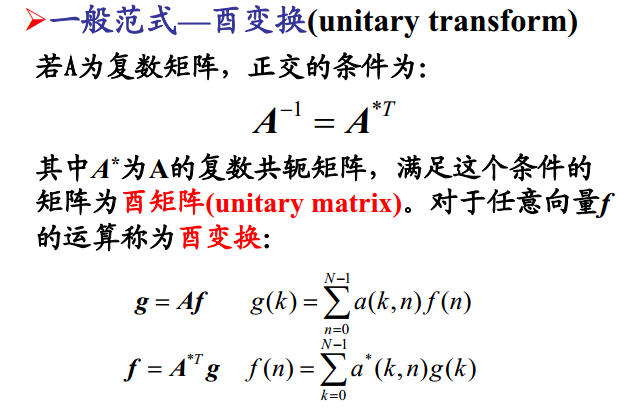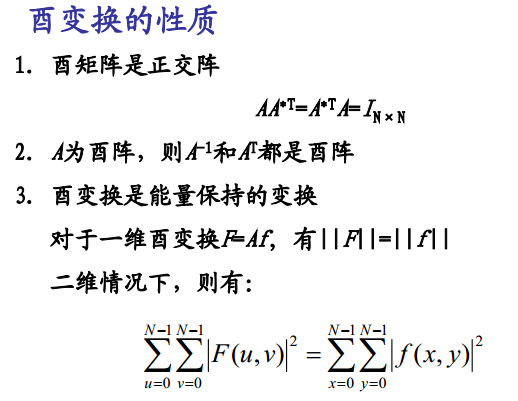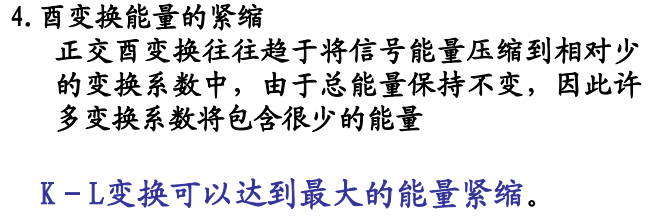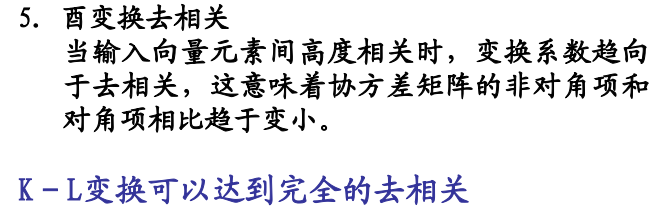p11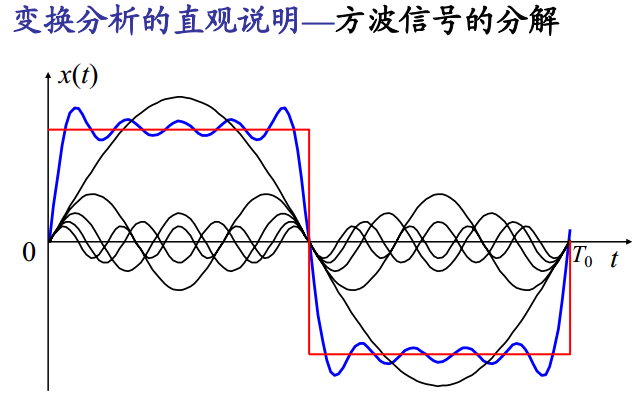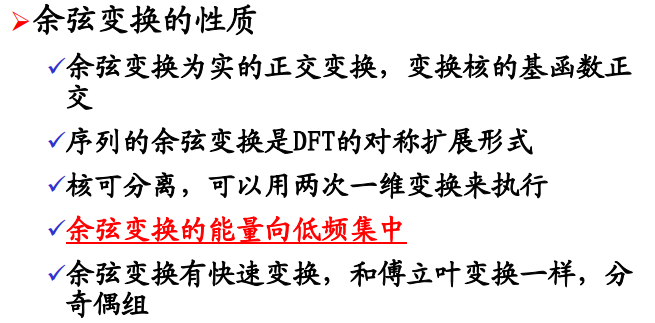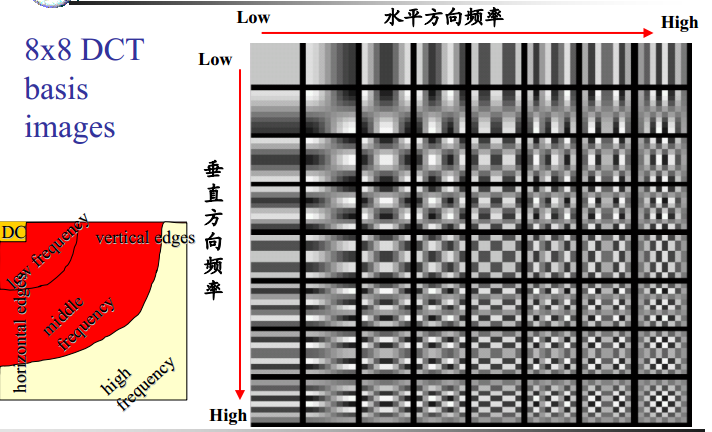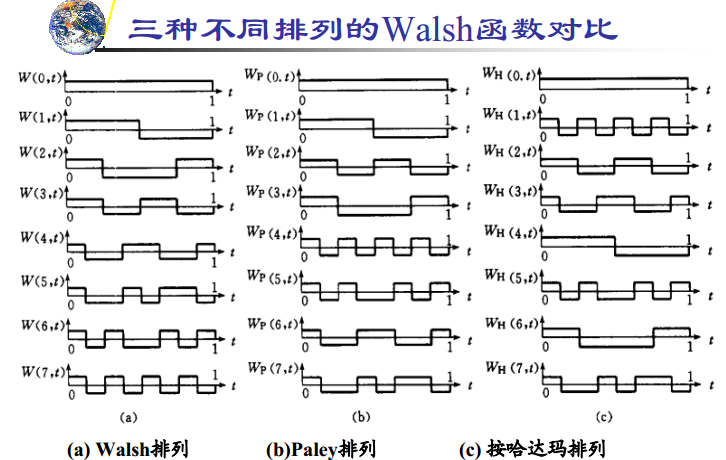8个函数满足正交性和完备性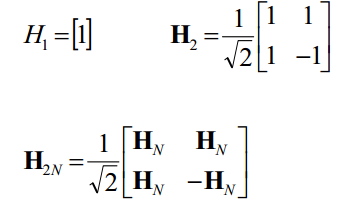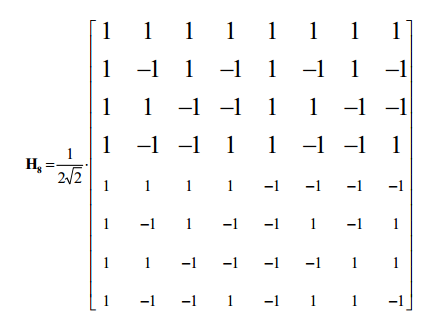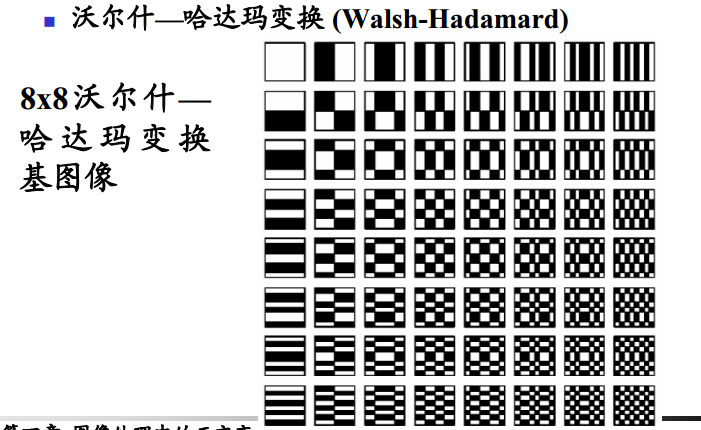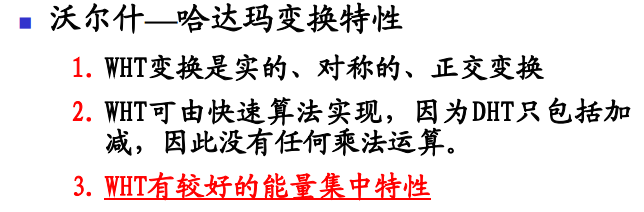K-L变换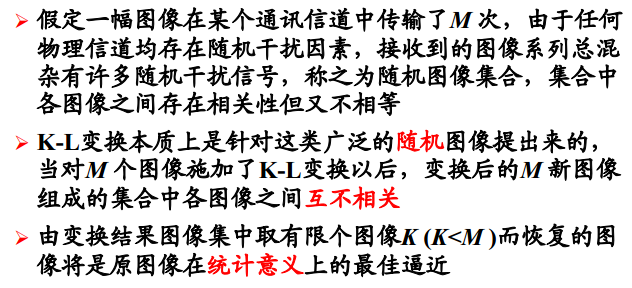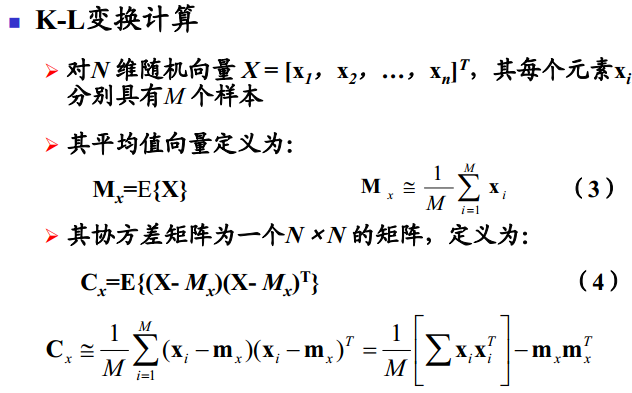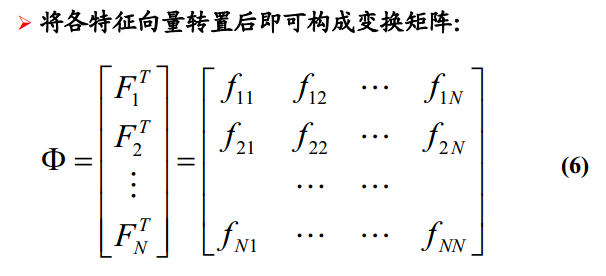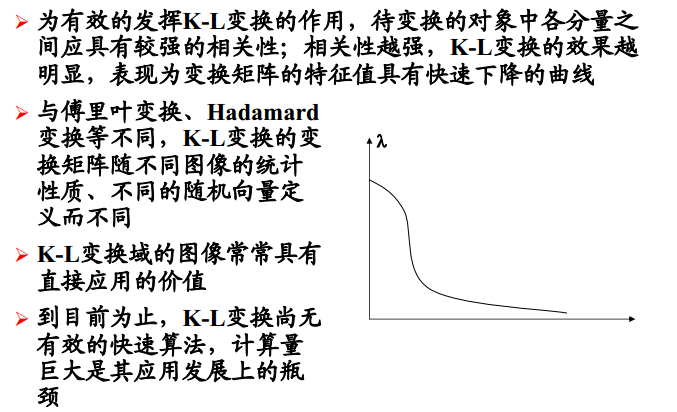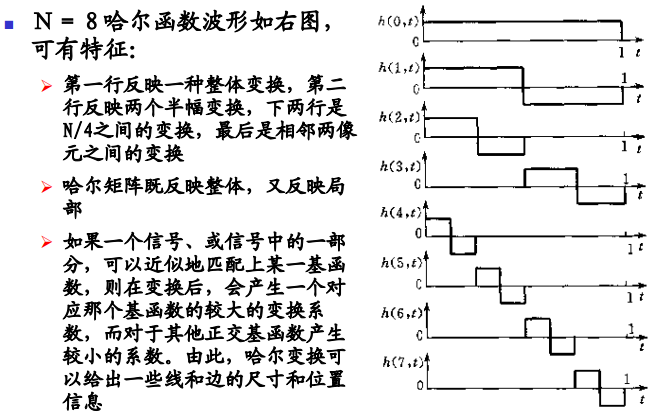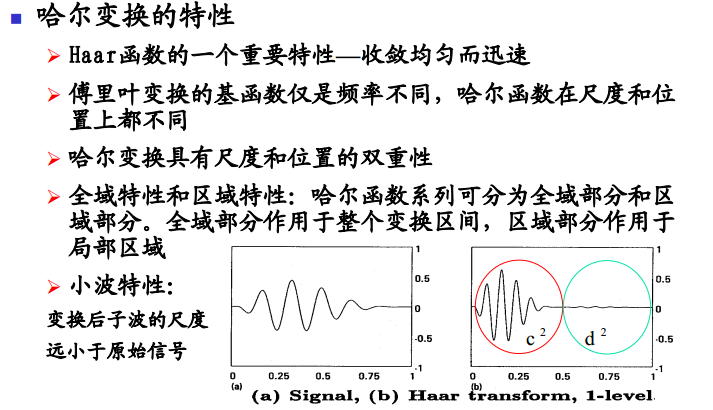p14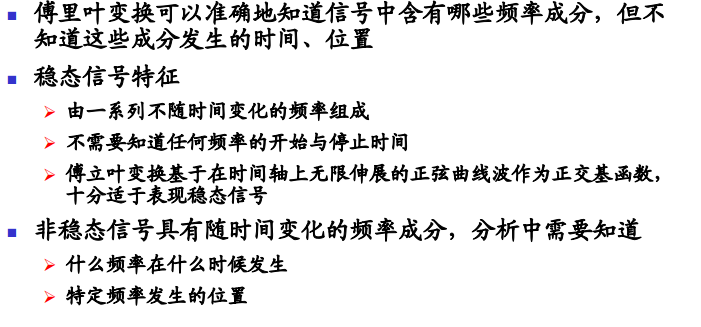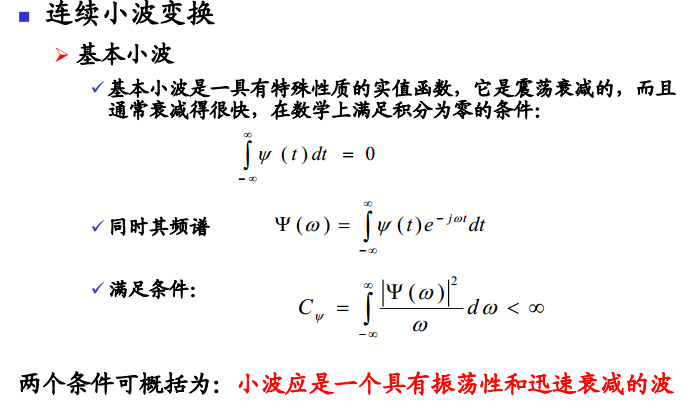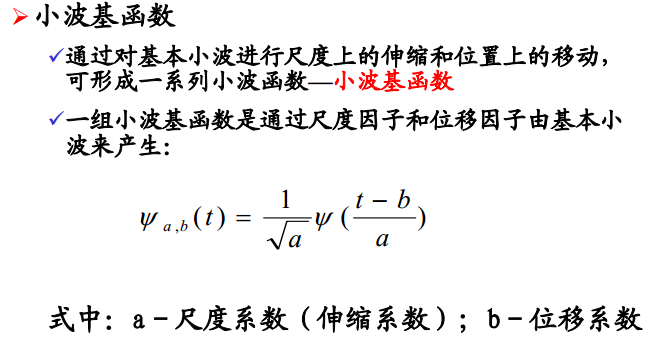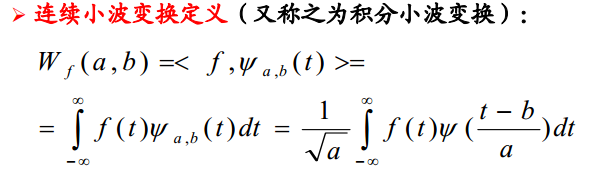Mexican Hat Wavelet

Haar Wavelet

Morlet Wavelet

Daubechies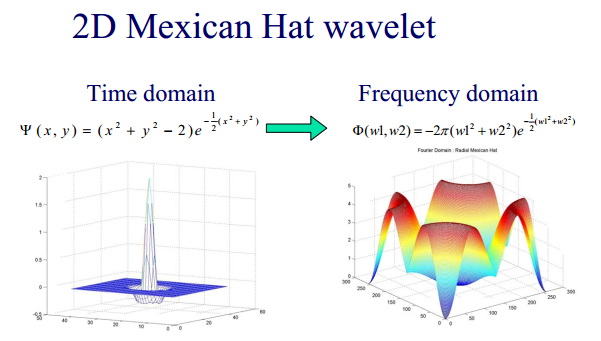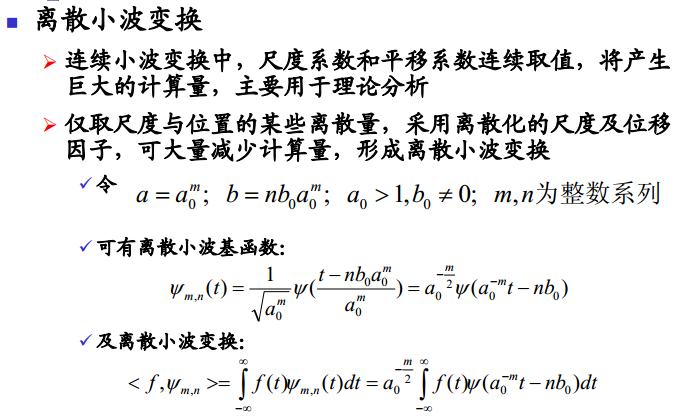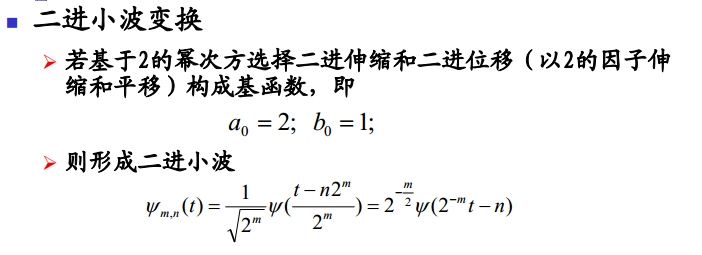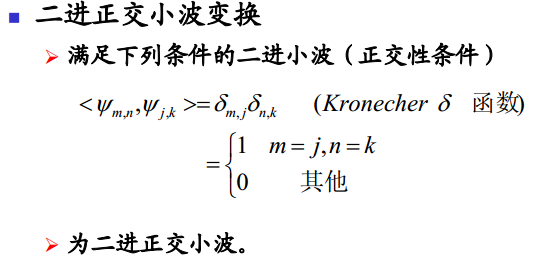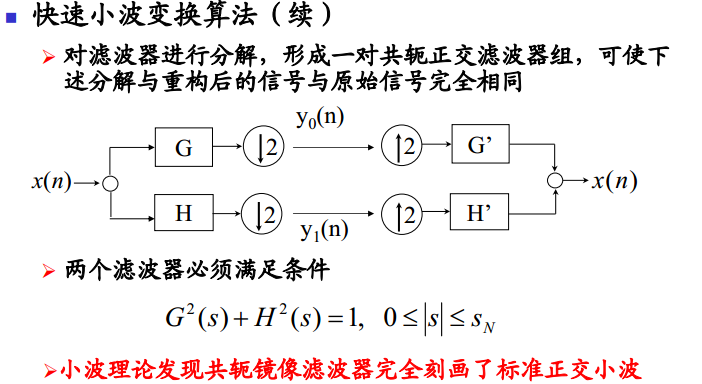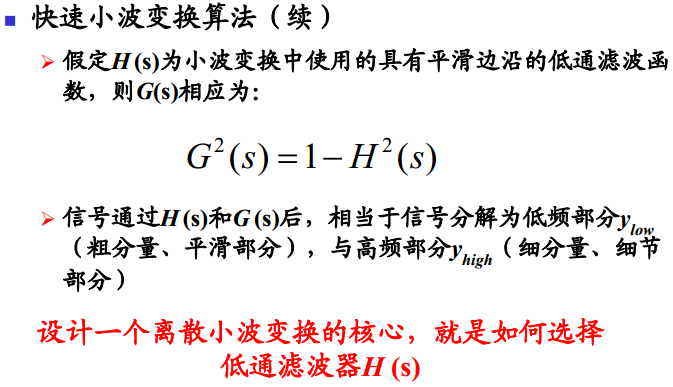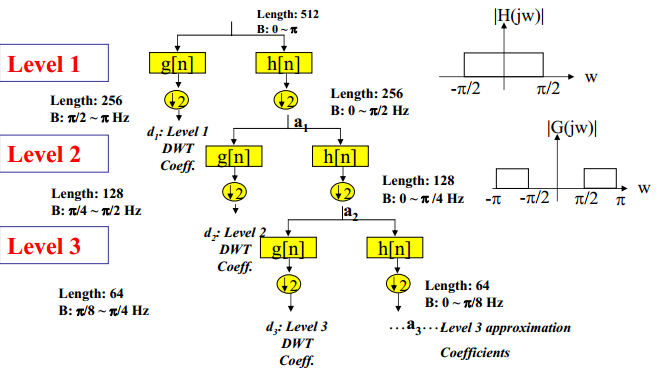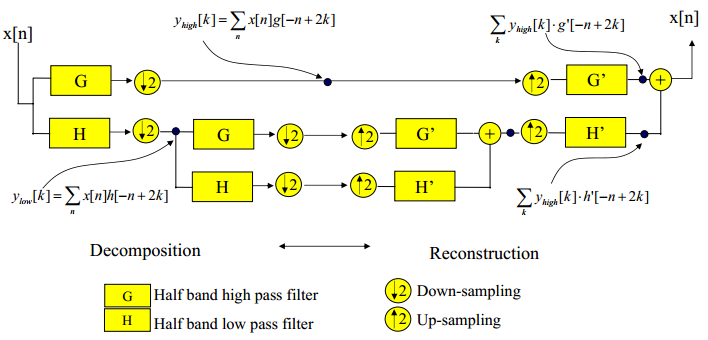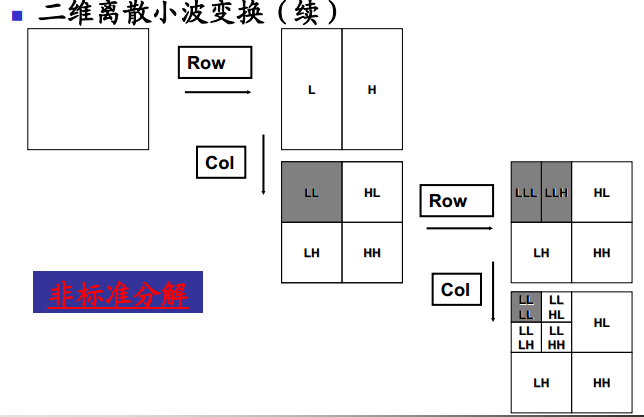2019-07-25 21:37:15 Arthur_Holmes 阅读数 195
• ###### 多角度带你编写更规范的黑盒测试用例

讲解方式 通过类比生活中的例子，轻松理解测试用例的设计方法。课程内容条理清晰，目标明确，由浅入深，环环相扣。重点部分进行额外梳理和总结，更易理解和吸收。 课程亮点 1，测试用例的要素讲解，让测试用例的编写更加规范 2、多种测试用例方法的学习，让用例设计更加全面。测试用例的设计方法：等价类、边界值、判定表、因果图、状态迁移图、场景法、正交实验法、错误推测法 3，多角度的案例实践，以理解各种方法的应用 课程内容 1、测试用例的定义和组成要素 2、测试用例的设计方法：等价类、边界值、判定表、因果图、状态迁移图、场景法、正交实验法、错误推测法 3：每种设计方法对应的案例讲解 适用人群 1、对软件测试感兴趣的在校生及应届生。 2、希望转行软件测试的在职人员。 3、希望巩固软件测试设计方法的测试同行。 4、对软件测试感兴趣的其他听众。

626 人正在学习 去看看 传智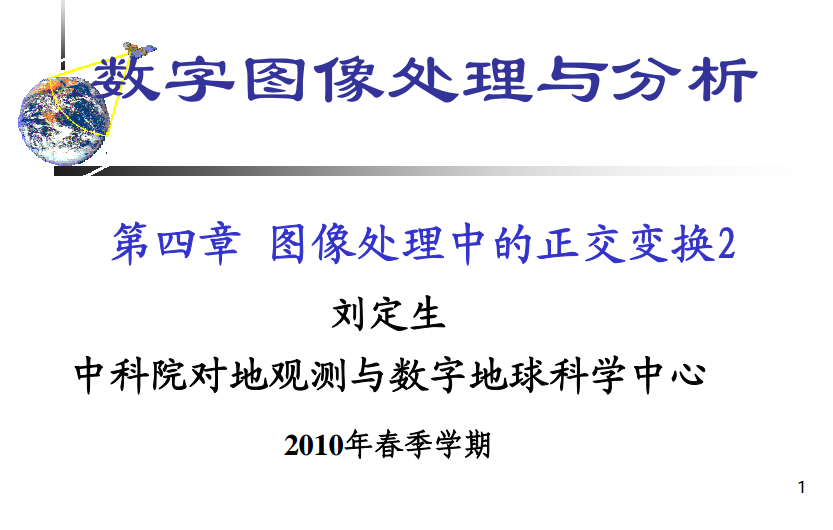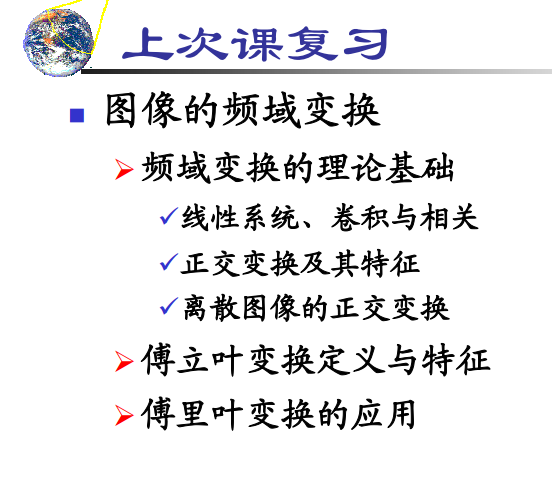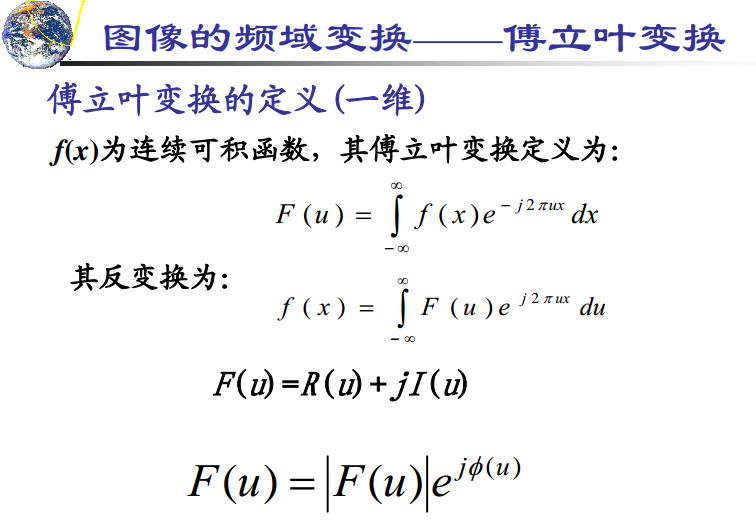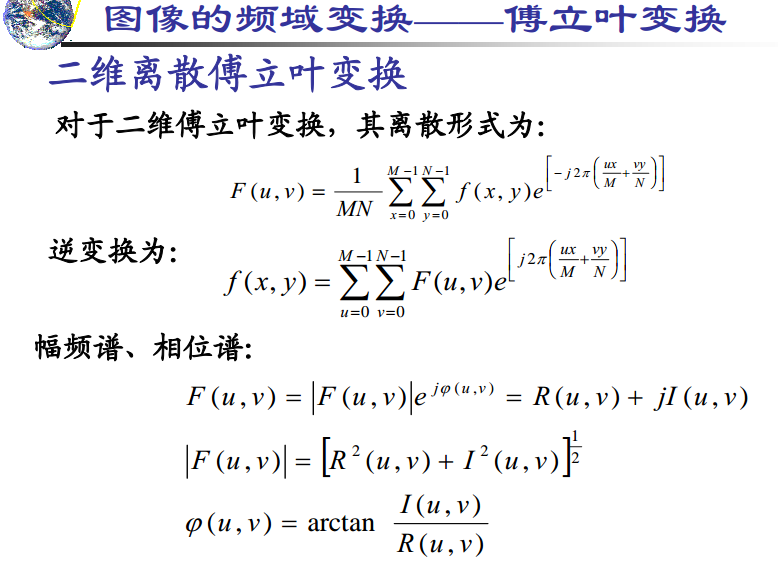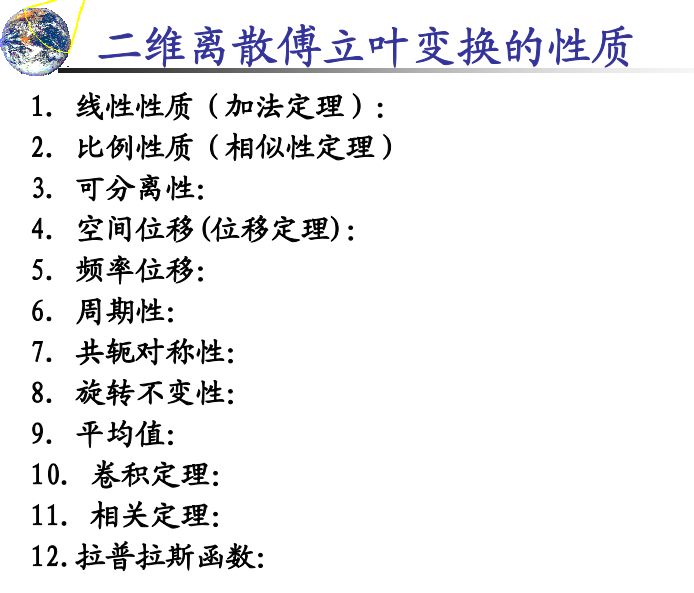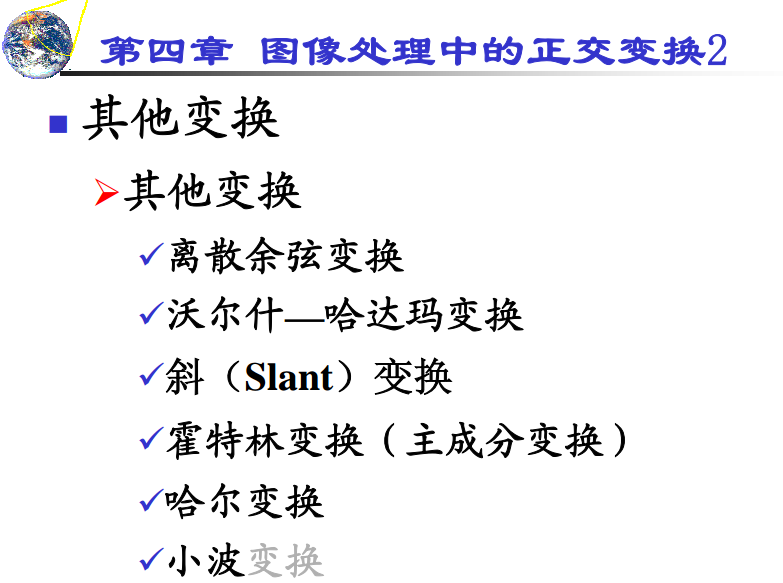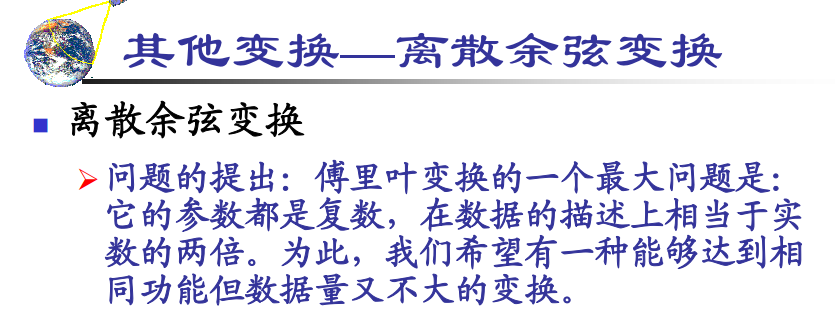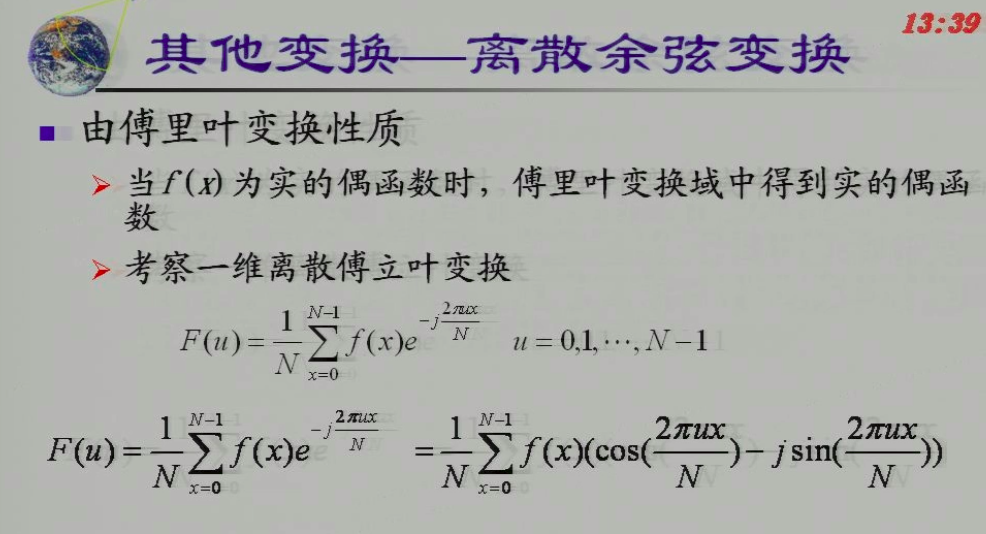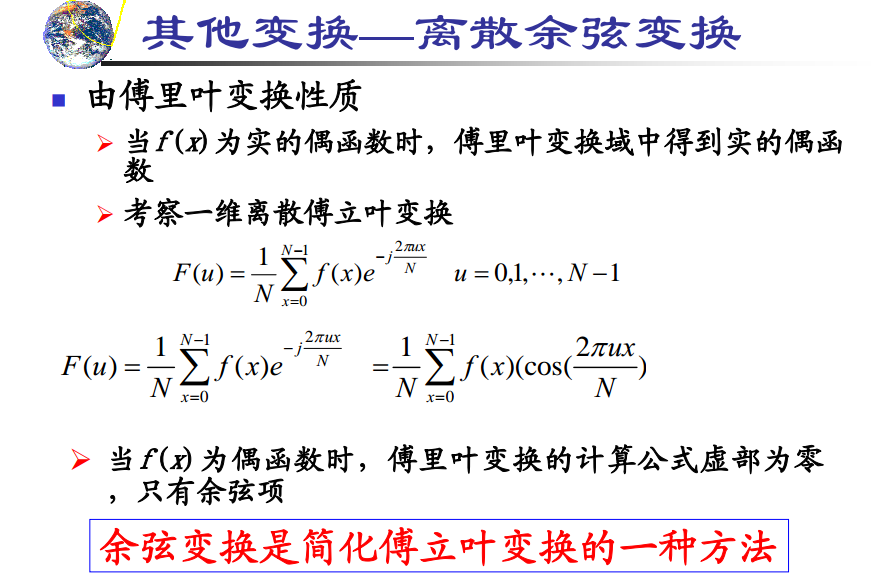### 实函数的要求已经达到，但是偶函数的要求比较难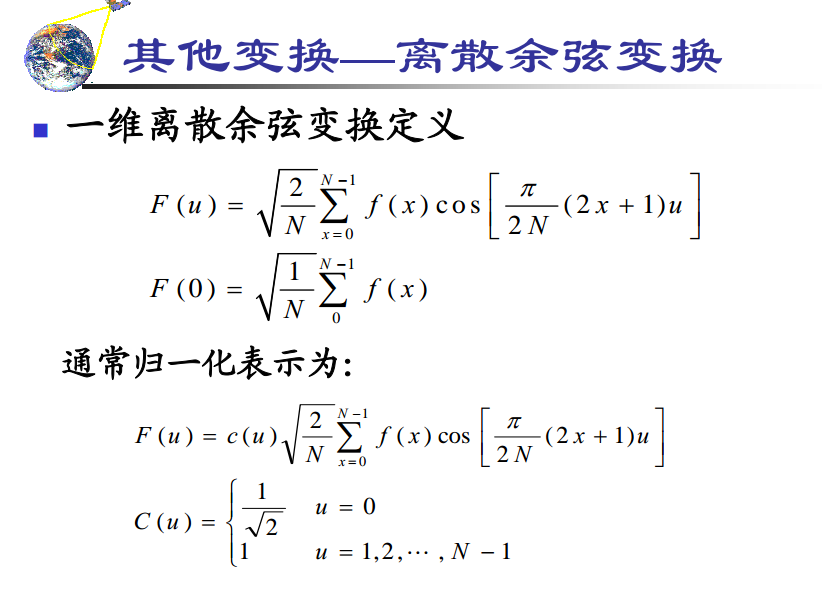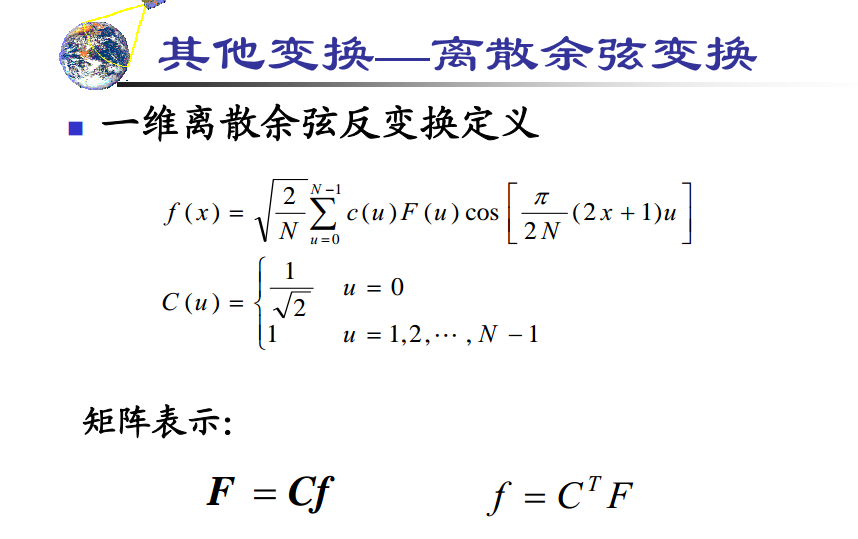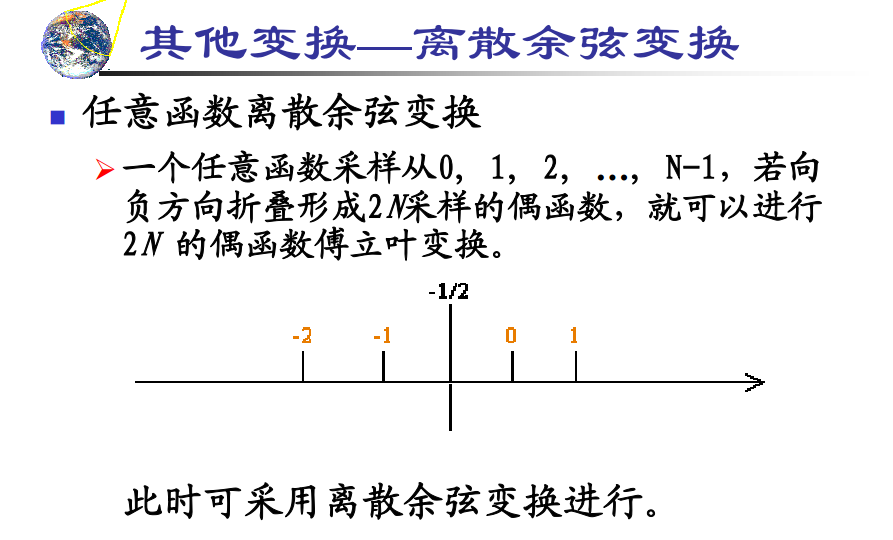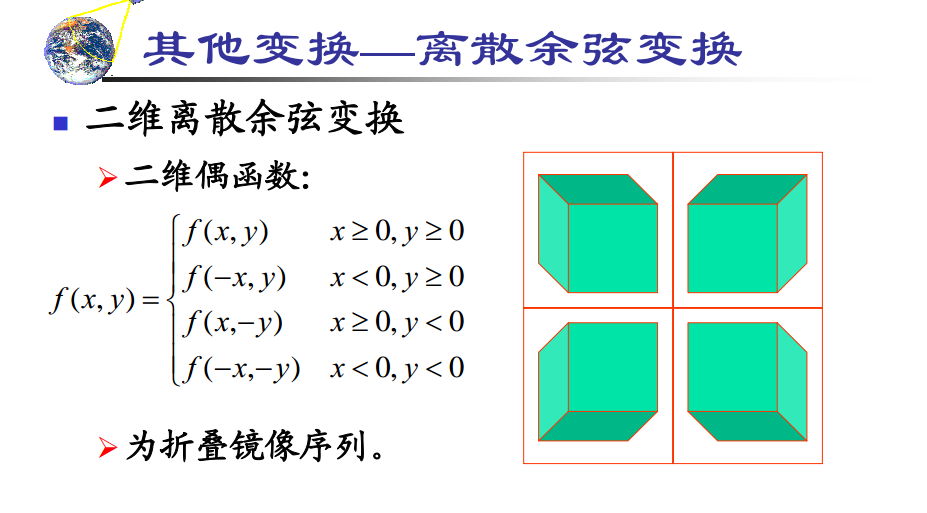### 假定具有这种对称性，真实情况比会有误差，但是误差可以忽略不计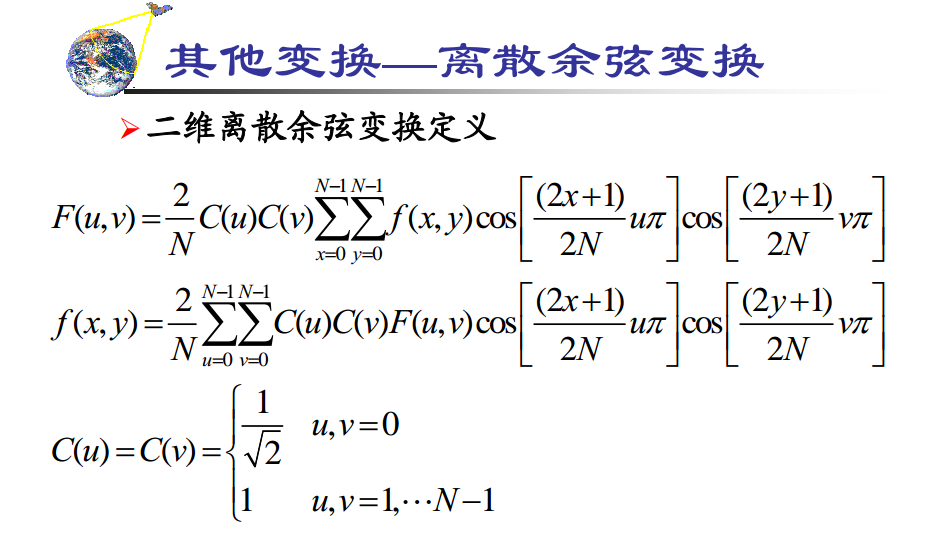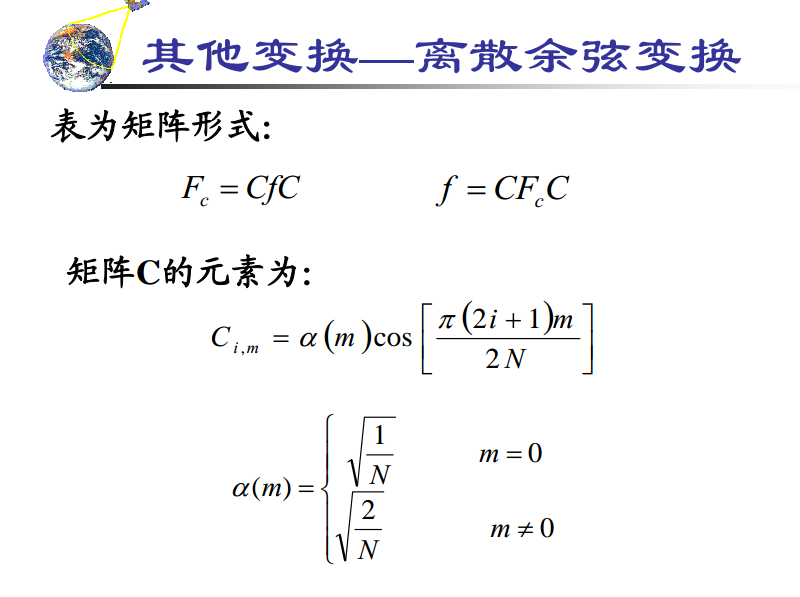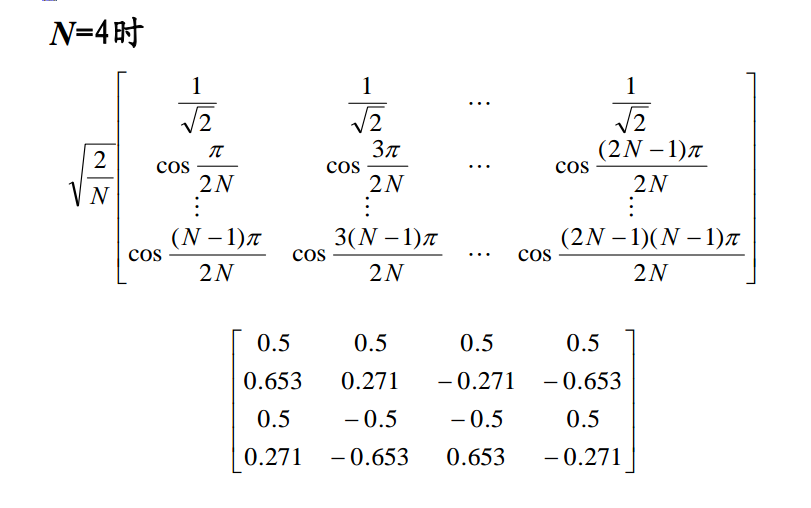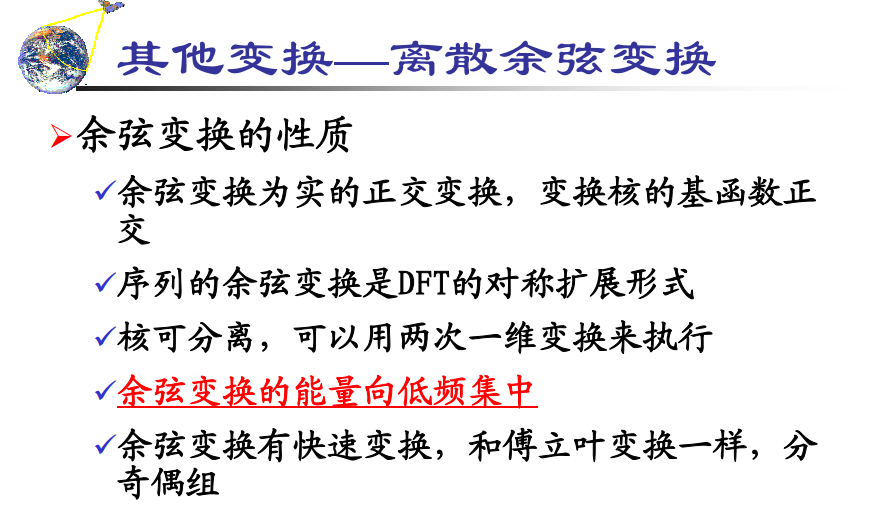### 余弦变换因为只有实变换，所以只有单个幅频谱，而且显然比傅里叶变换更加集中（并且在一个点，而不是四个点）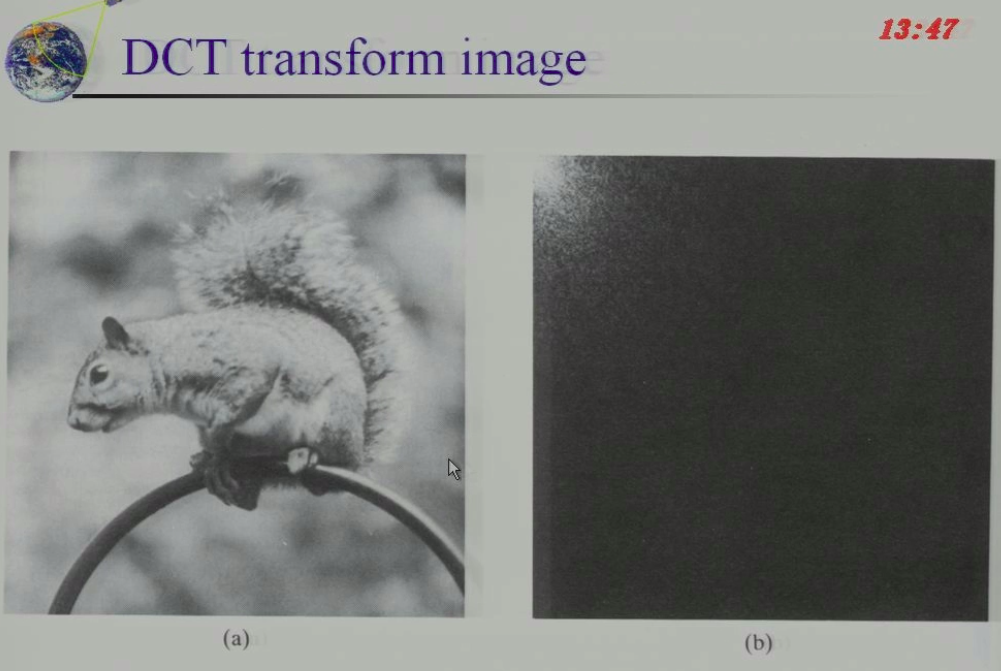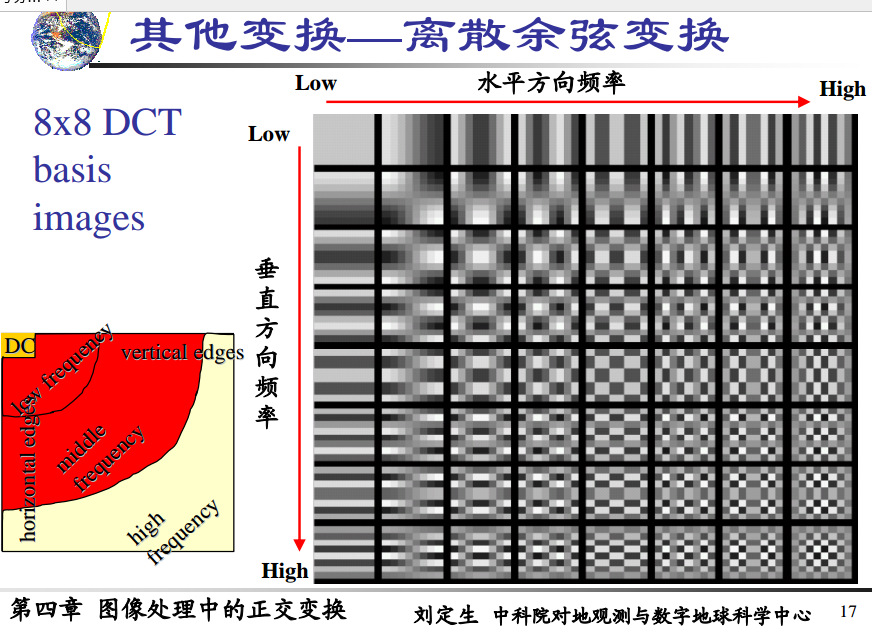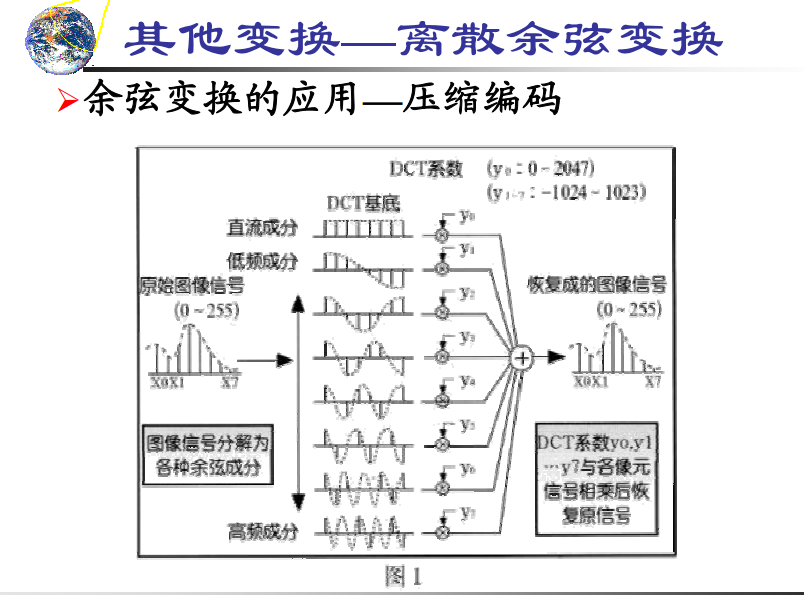### JPG等都用的是余弦变换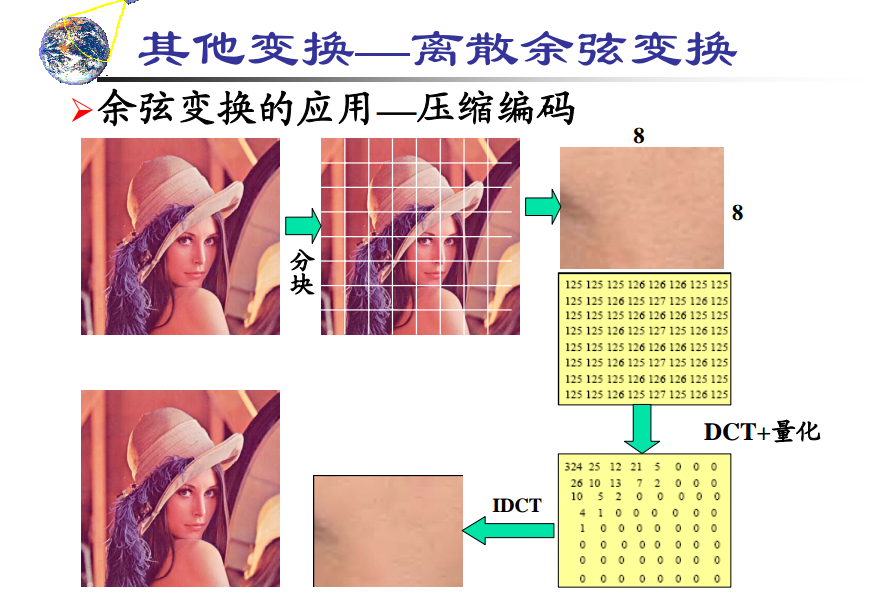### 为什么分成小块，因为大块大大增加运算量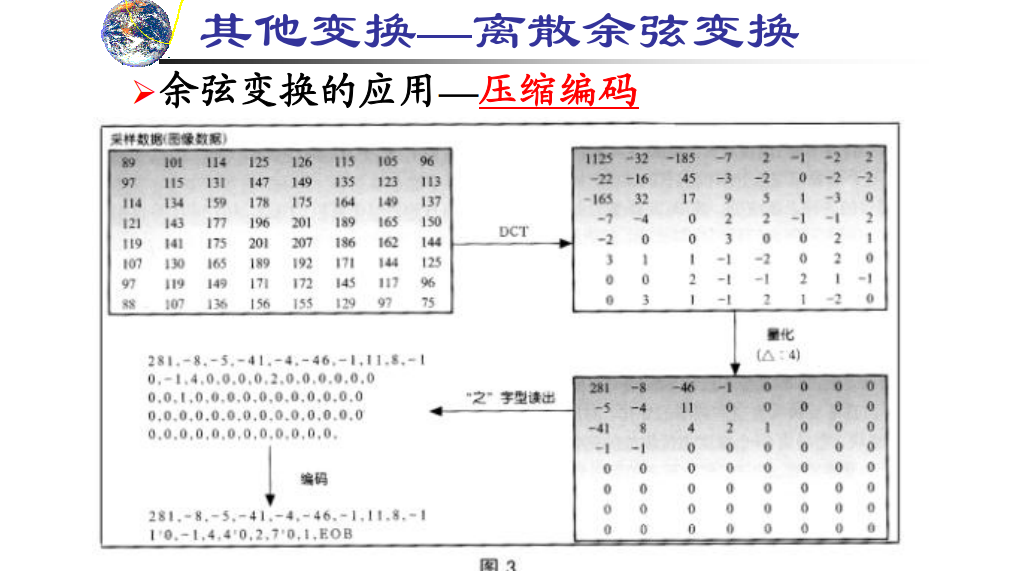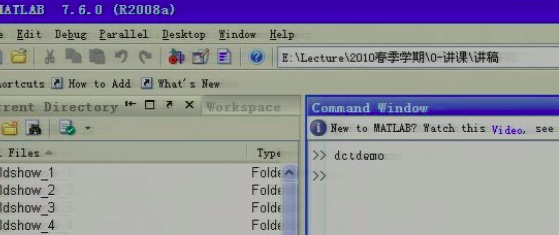### MATLAB调用demo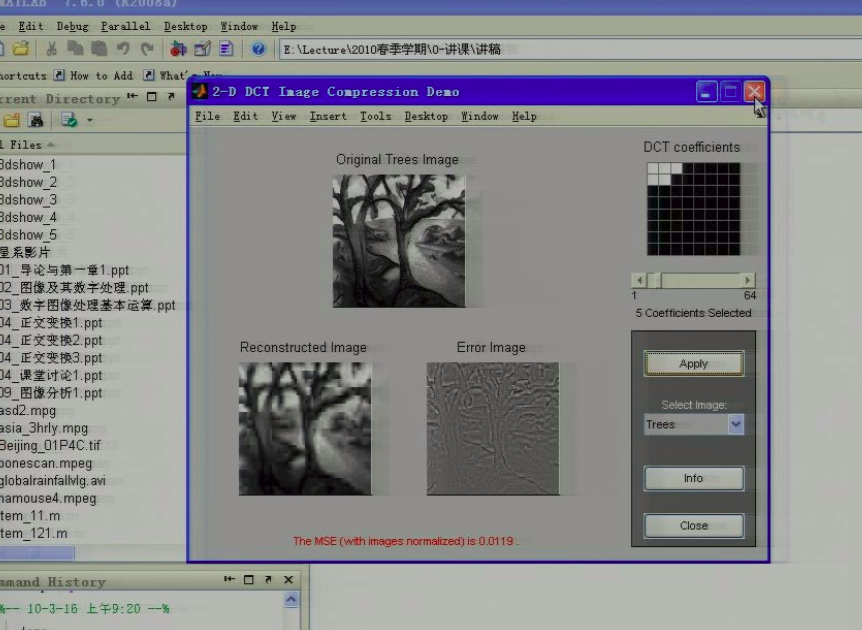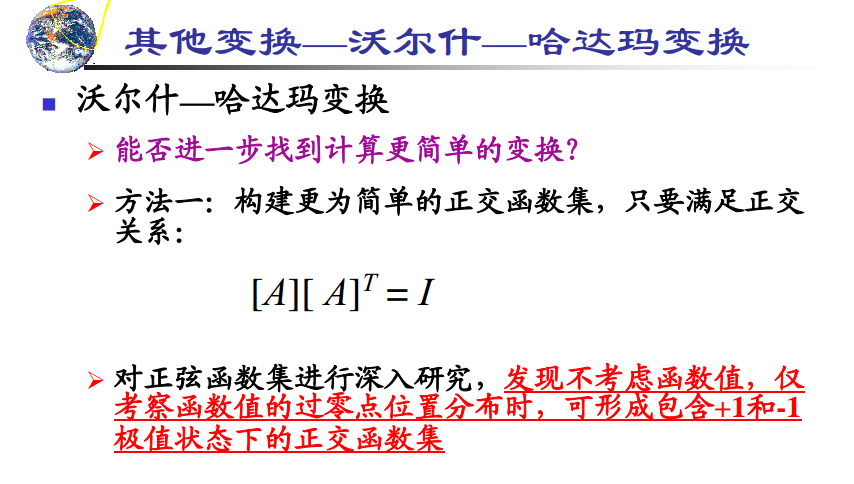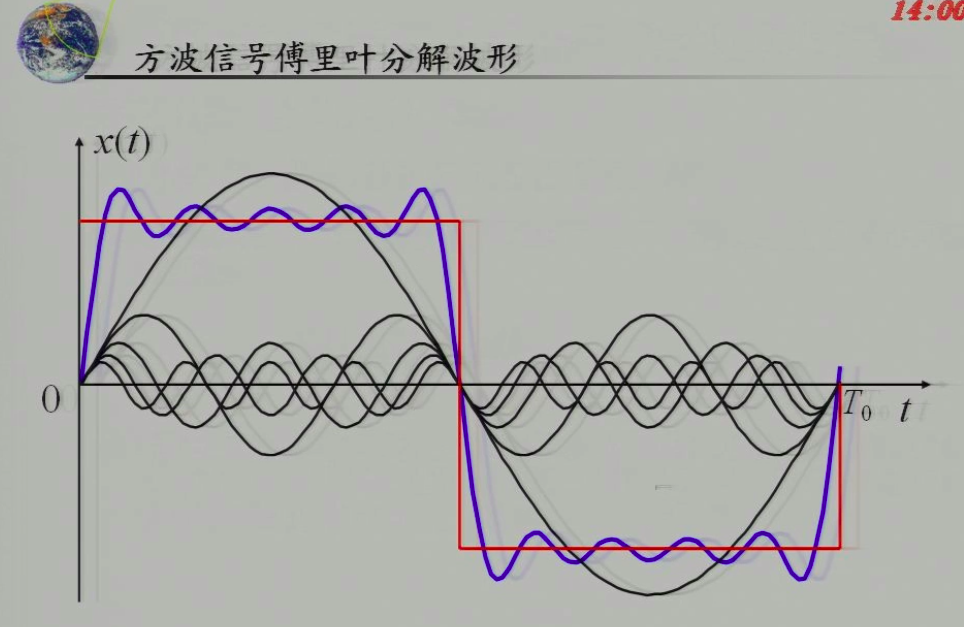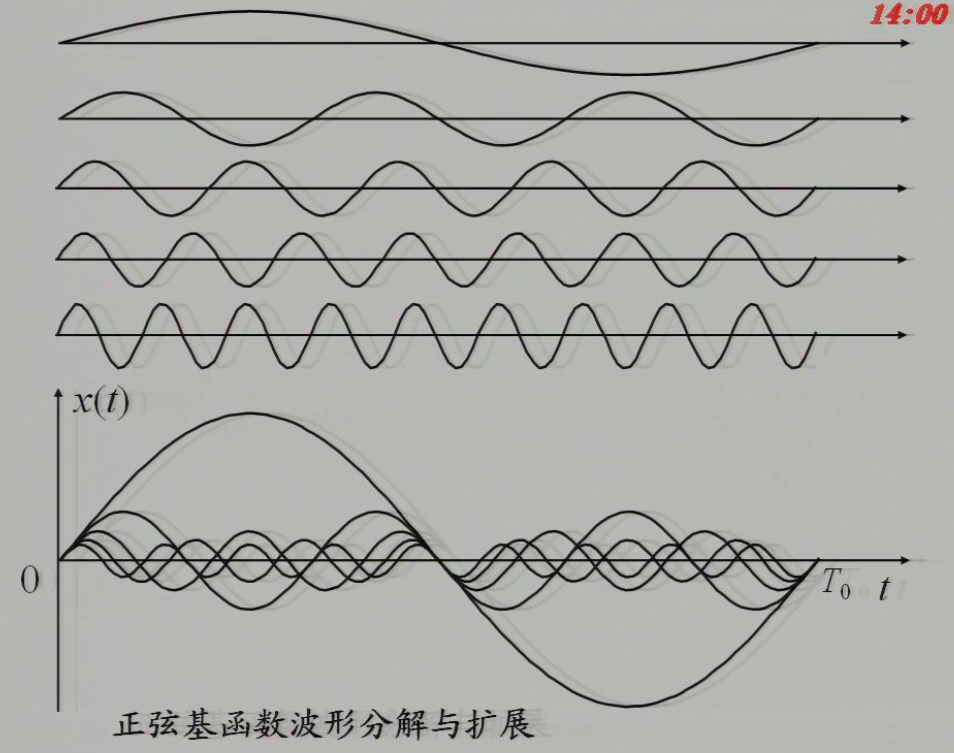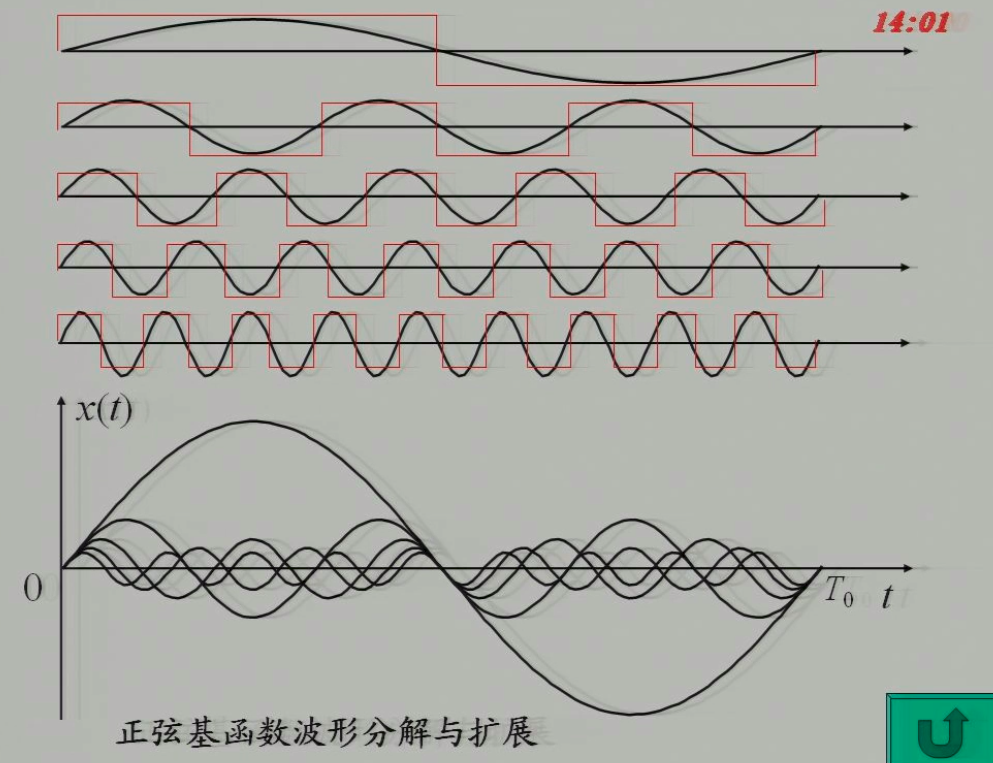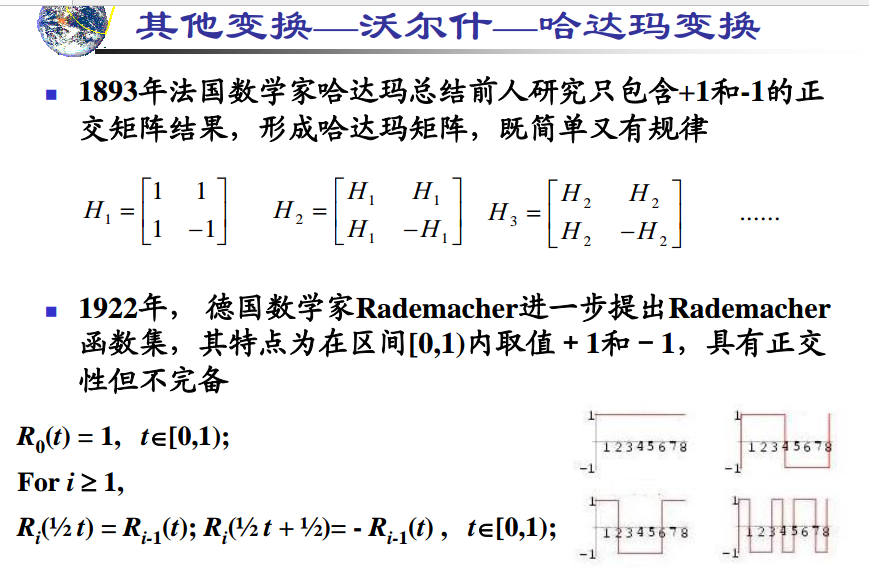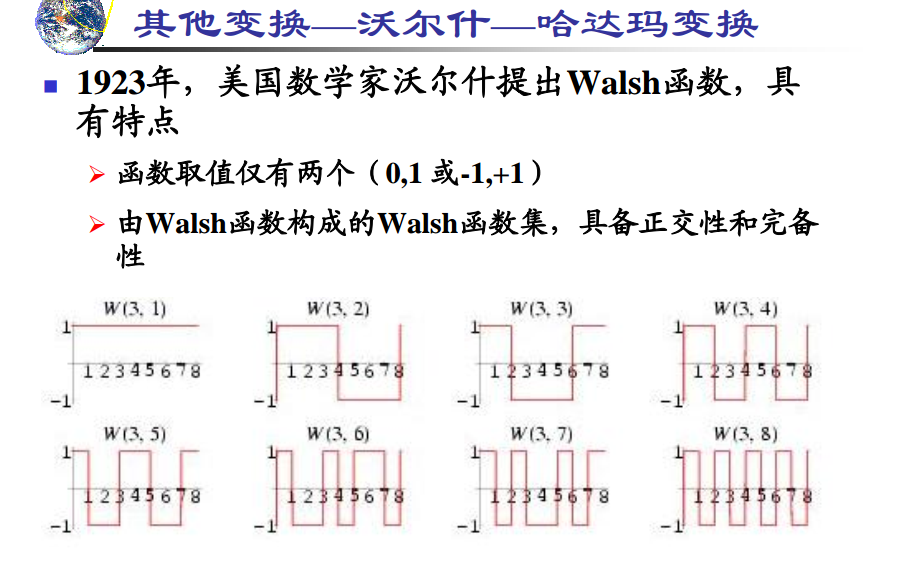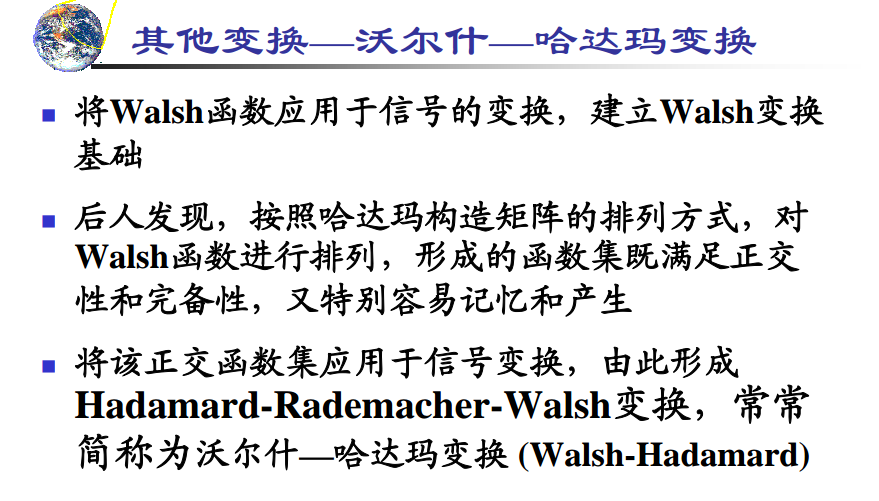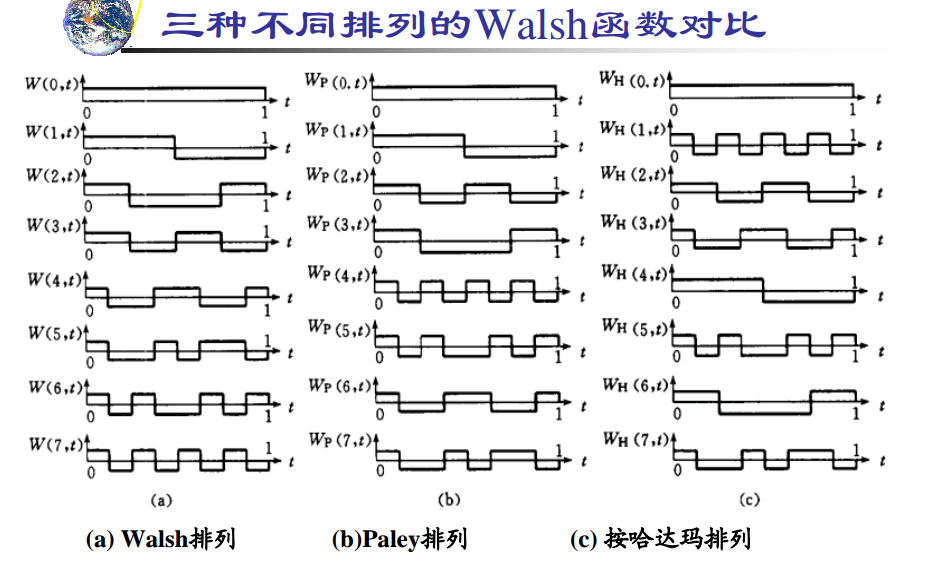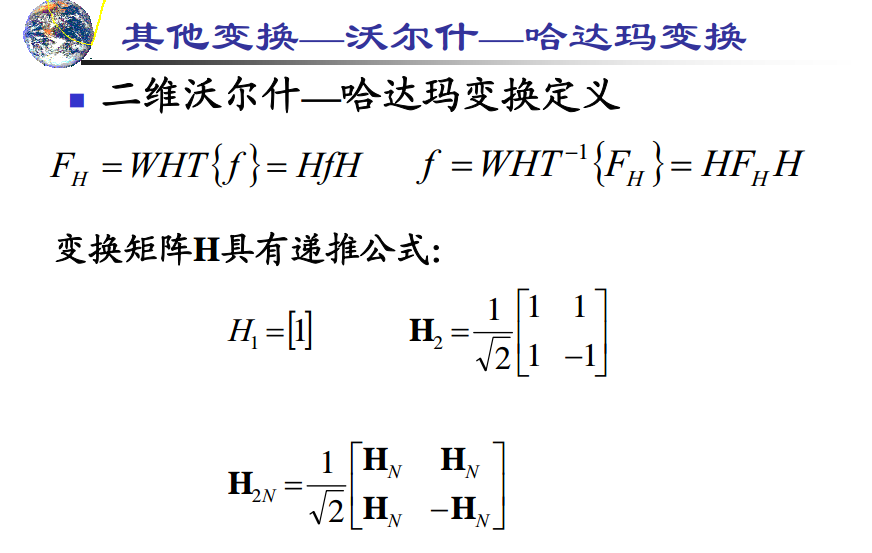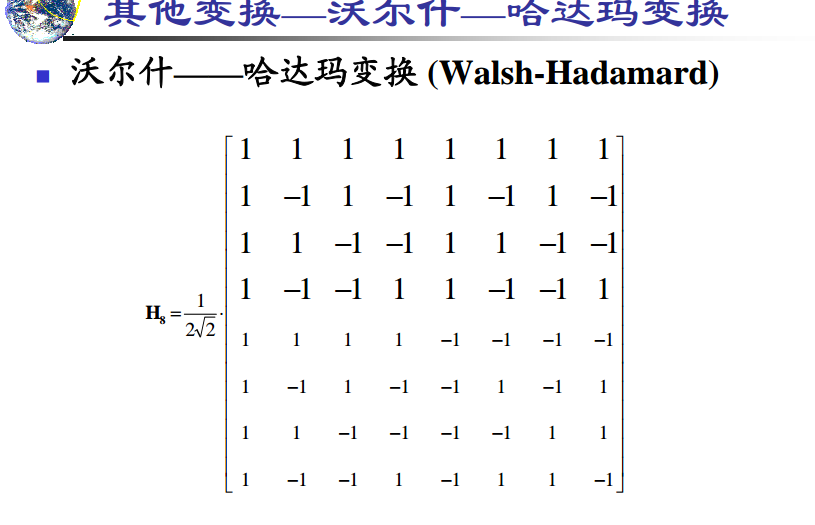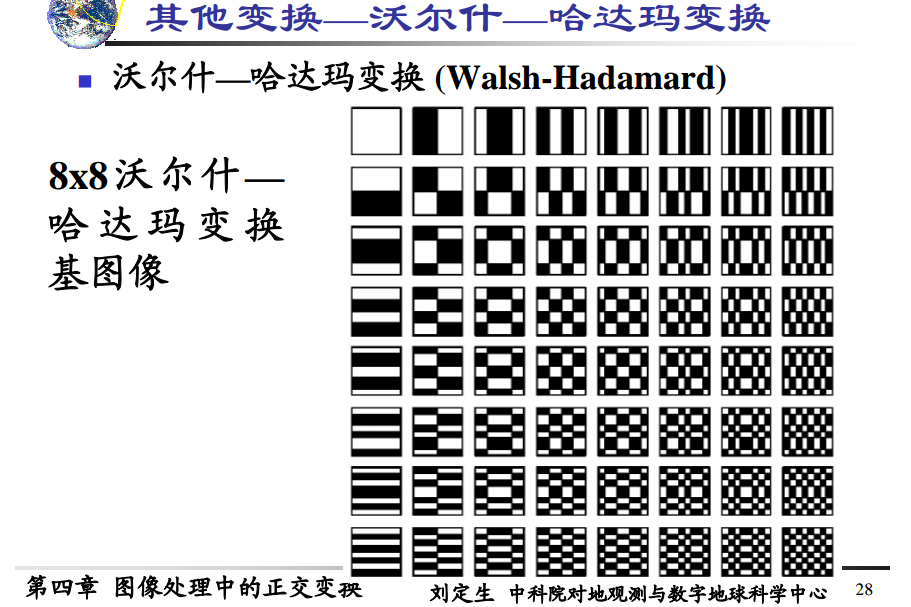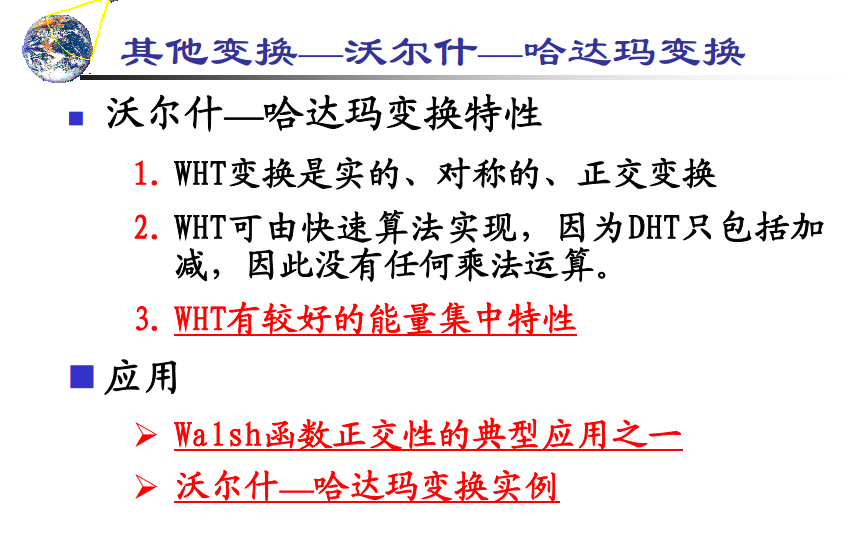### 哈达玛变换是对离散余弦变换的近似，所以比离散余弦的集中特性要差一点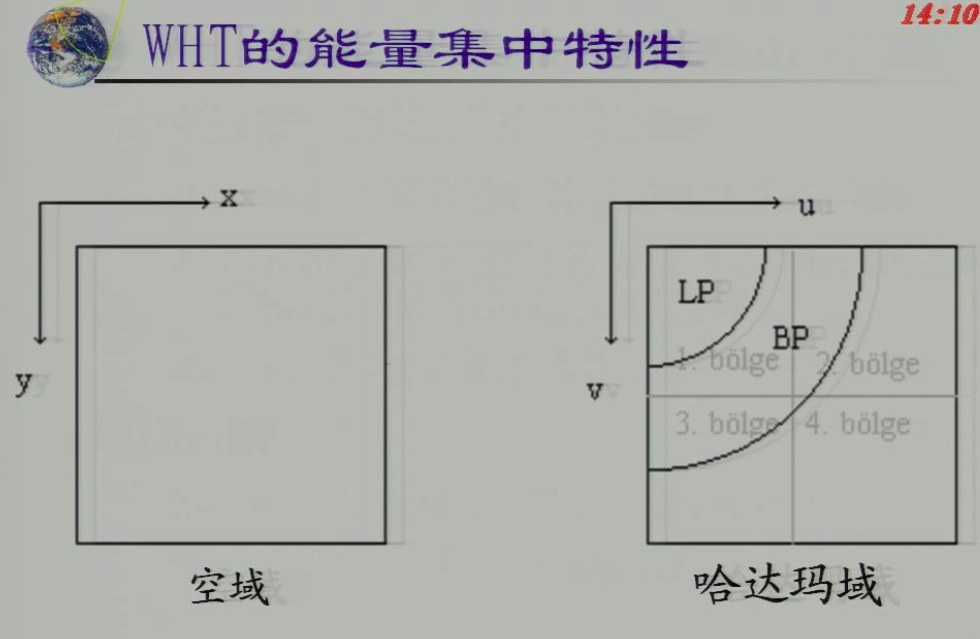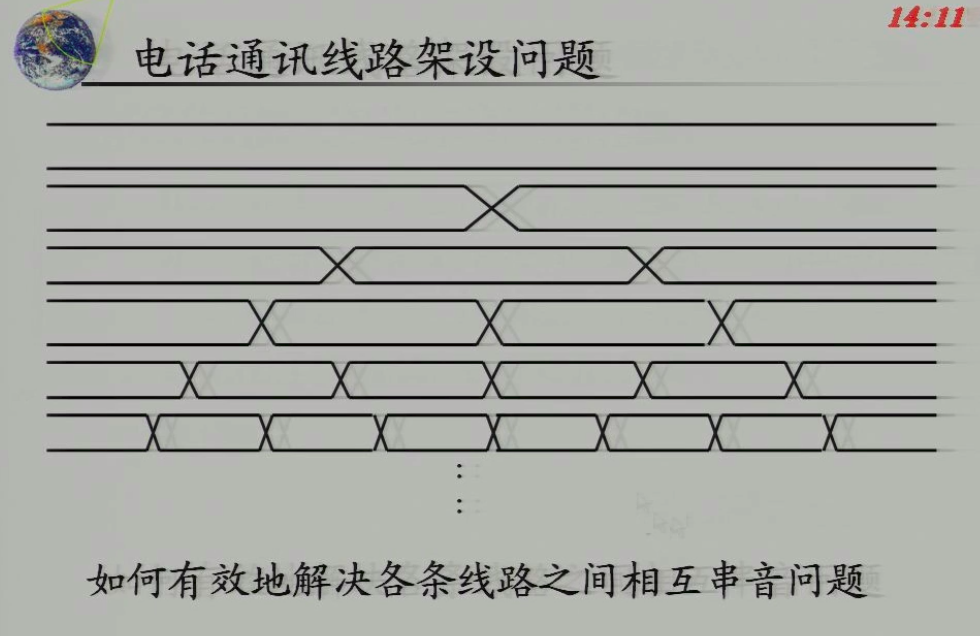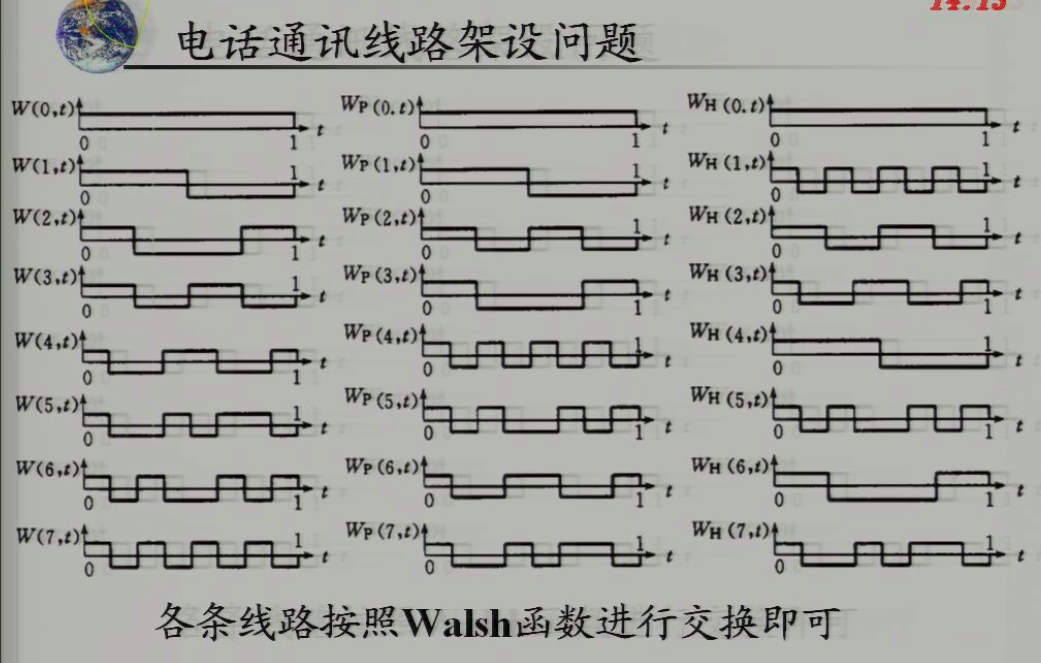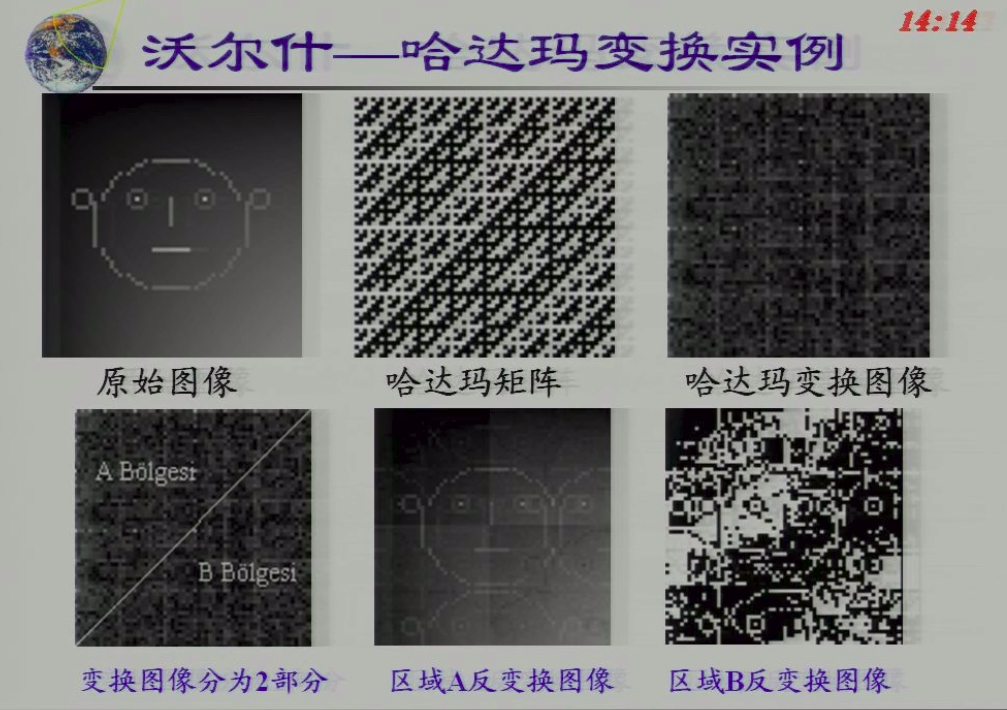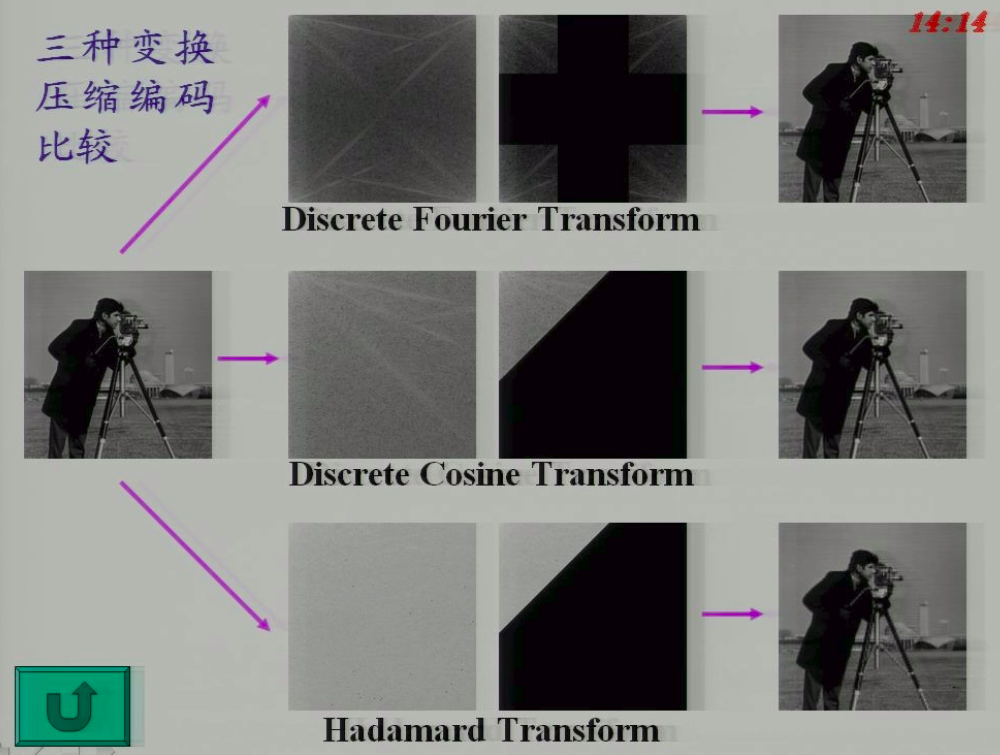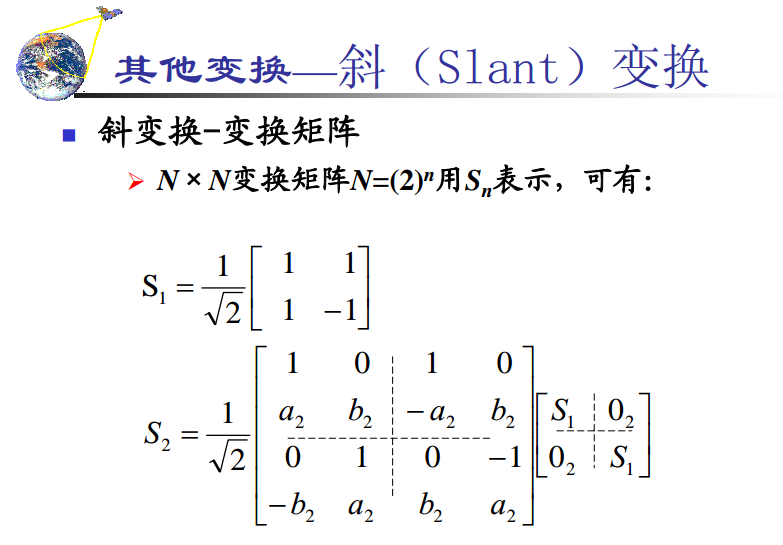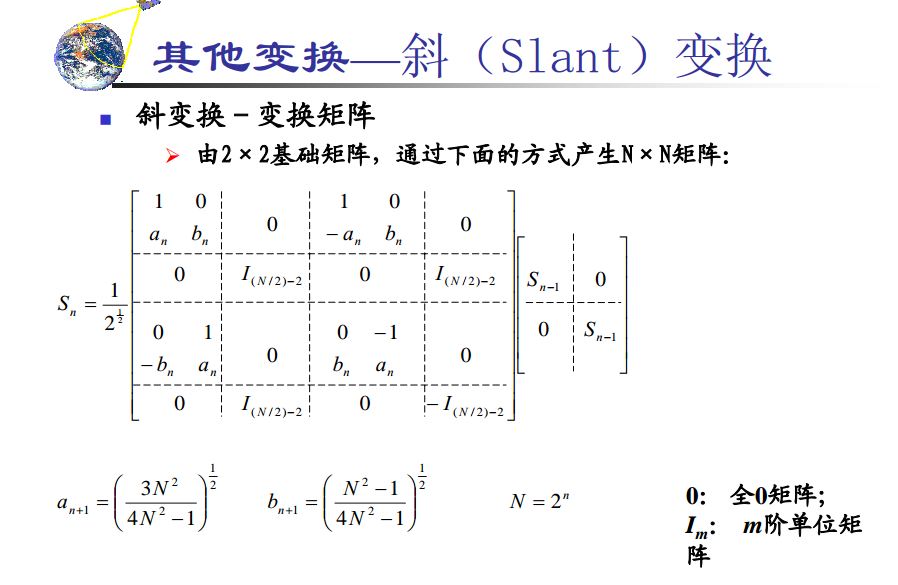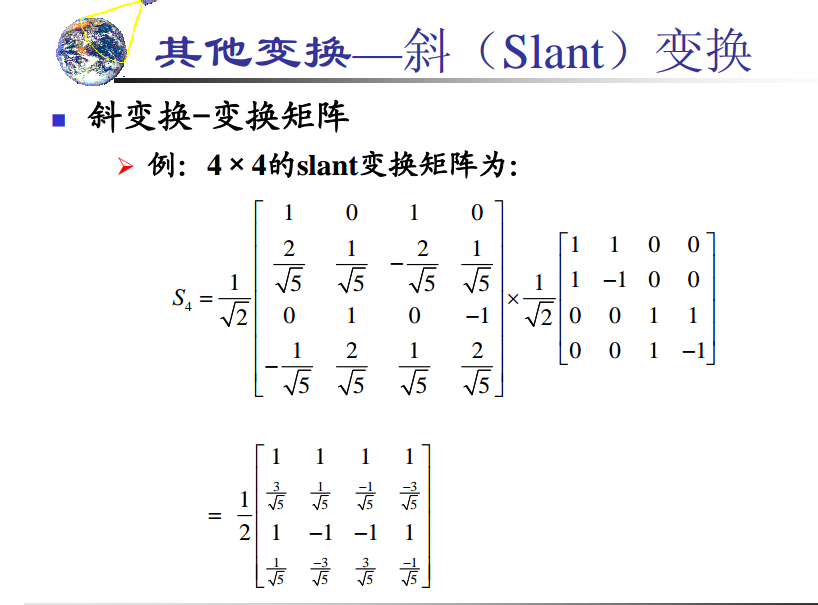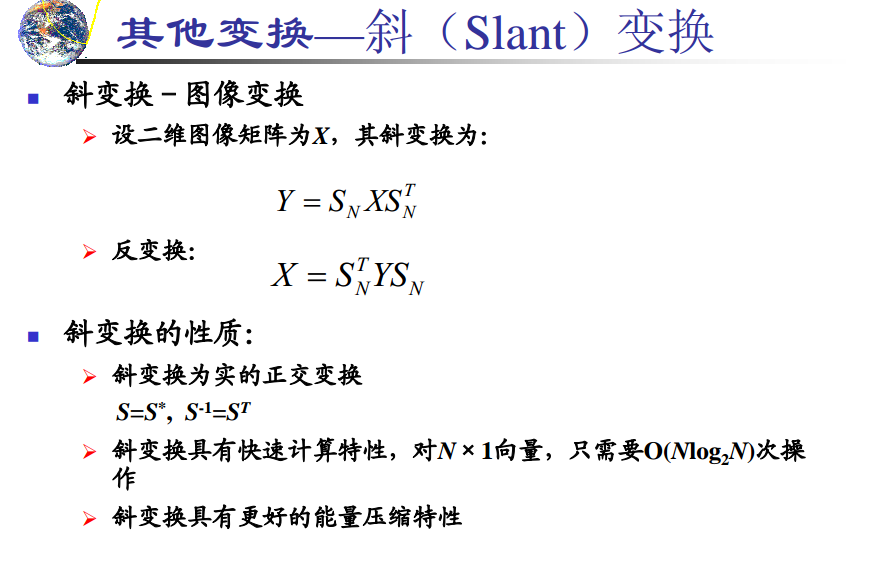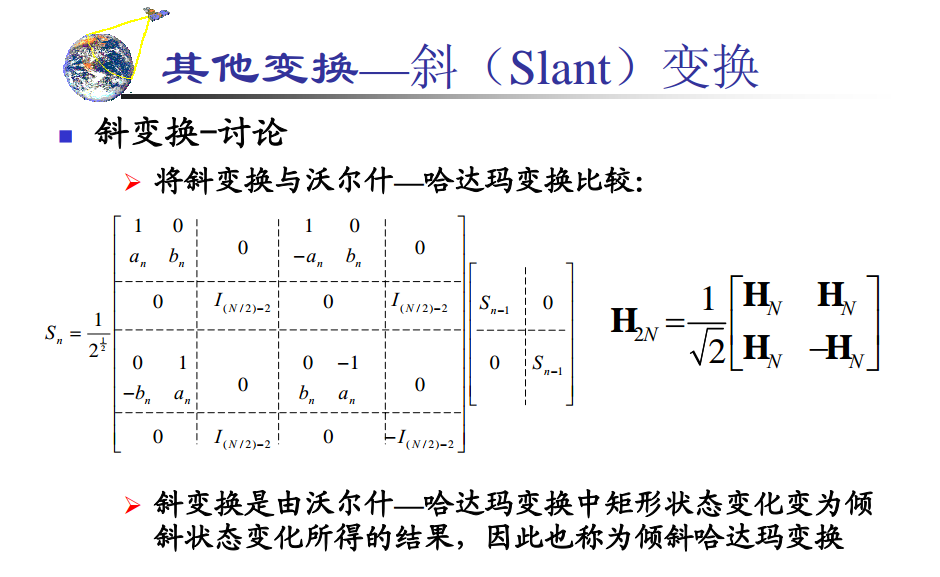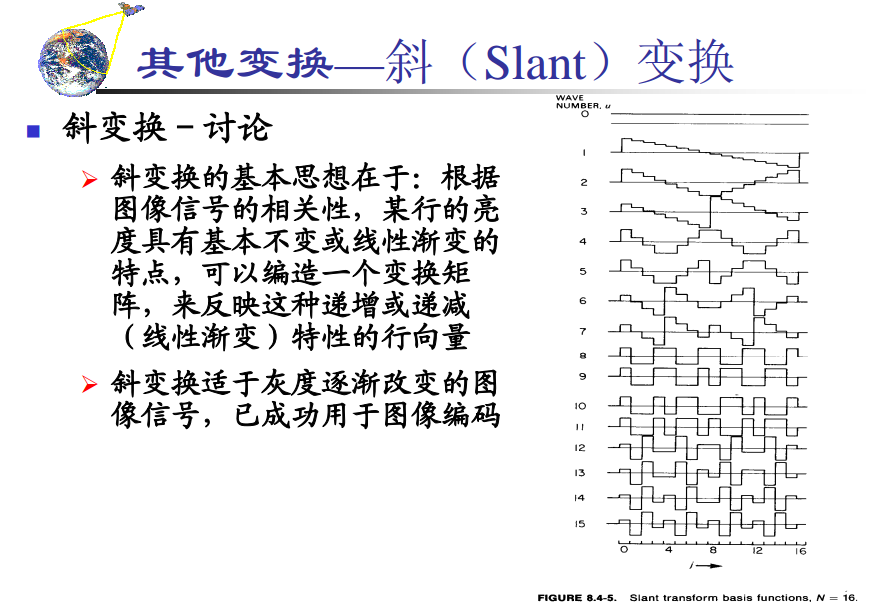2019-07-24 15:27:46 baishuiniyaonulia 阅读数 785
• ###### 多角度带你编写更规范的黑盒测试用例

讲解方式 通过类比生活中的例子，轻松理解测试用例的设计方法。课程内容条理清晰，目标明确，由浅入深，环环相扣。重点部分进行额外梳理和总结，更易理解和吸收。 课程亮点 1，测试用例的要素讲解，让测试用例的编写更加规范 2、多种测试用例方法的学习，让用例设计更加全面。测试用例的设计方法：等价类、边界值、判定表、因果图、状态迁移图、场景法、正交实验法、错误推测法 3，多角度的案例实践，以理解各种方法的应用 课程内容 1、测试用例的定义和组成要素 2、测试用例的设计方法：等价类、边界值、判定表、因果图、状态迁移图、场景法、正交实验法、错误推测法 3：每种设计方法对应的案例讲解 适用人群 1、对软件测试感兴趣的在校生及应届生。 2、希望转行软件测试的在职人员。 3、希望巩固软件测试设计方法的测试同行。 4、对软件测试感兴趣的其他听众。

626 人正在学习 去看看 传智

### 图像正交变换的本质

1. 正弦余弦型变换
2. 方波型变换
3. 基于特征向量的变换

K-L变换 在许多意义下是最佳的，但是无快速算法，对彩色图像或人脸图像这类特征图像十分有效
##### 冲激函数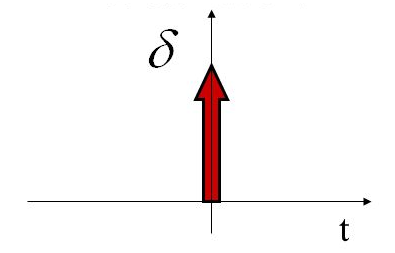### 一维正交变换

##### 线性变换

$t$为一个$N*1$向量，$T$为一个$N*N$矩阵，$T$作用于列向量$f(x)$得到列向量$F$，这就定义了向量$t$的一个线性变换，表达为：$F(\mu)=\sum_{x=0}^{N-1}f(x)g(x,\mu),\mu\in[0,N-1]$其矩阵形式为：$F=Tf(x)$其中，$f(x)$称为冲激$g(t,\mu)$称为正变换核函数冲激响应，矩阵$T$称为变换的核矩阵变换矩阵

$\begin{bmatrix} y_1 \\ y_2 \\ \end{bmatrix}=\begin{bmatrix} \cos\theta & -\sin\theta\\ \sin\theta & \cos\theta\\ \end{bmatrix}\begin{bmatrix} x_1 \\ x_2 \\ \end{bmatrix}$可表示为：$y=Tx$其中$T=\begin{bmatrix} \cos\theta & -\sin\theta\\ \sin\theta & \cos\theta\\ \end{bmatrix}$，该矩阵表达式的展开式为:$\begin{cases} y_1=x_1\cos\theta-x_2\sin\theta\\ y_2=x_1\sin\theta+x_2\cos\theta \end{cases}$若将$T$抽象表达为$T=\begin{bmatrix} a_{11} & a_{12}\\ a_{21} & a_{22}\\ \end{bmatrix}$，则展开式可以表达为：$\begin{cases} y_1=x_1a_{11}+x_2a_{12}\\ y_2=x_1a_{21}+x_2a_{22} \end{cases}$，简化得：$y_i=\sum_{i=1}^{2}x_ia_{j,i}，j\in[1,2]$

##### 酉变换与正交性

$T$的逆变换等于其复数矩阵共轭的转置时，称该线性变换为酉变换，表示为：$T^{-1}=(T^{*})^T=(T^{*})'$$TT^{-1}=T(T^*)'=E$

$T$为实数矩阵时，实数的共轭等于实数本身，此时酉变换就称为正交变换，表示为：$T^{-1}=T'$，于是显然有：$TT'=E$

##### 完备性

$f(x)$是平方可积的分段连续函数，$\epsilon$表示无穷小量时，若总存在一个正整数$N$使得$\int_{-\infty}^{\infty}|f(x)-\hat f(x)|^2\text{d}x < \epsilon$其中$\hat f(x)$$f(x)$的估计值：$\hat f(x)=\sum_{n=0}^{N-1}a_n\mu_n(x)$则称$\mu_n(x)$函数集是完备的。完备则意味着定义域中任何$f(x)$均能用$\mu_n(x)$函数集表示。

### 二维正交变换

##### 二维离散线性变换

$F(\mu,\nu)=\sum_{x=0}^{N-1}\sum_{y=0}^{N-1}f(x,y)g(x,y,\mu,\nu),0\leqslant \mu,\nu\leqslant N-1$$f(x,y)=\sum_{\mu=0}^{N-1}\sum_{\nu=0}^{N-1}F(\mu,\nu)h(x,y,\mu,\nu),0\leqslant x,y\leqslant N-1$其中，$g(x,y,\mu,\nu)$称为正变换核函数$N^2*N^2$阶的正变换核矩阵$h(x,y,\mu,\nu)$称为逆变换核函数$N^2*N^2$阶的逆变换核矩阵

• 首先沿$f(x,y)$$y$方向做一维变换，有$F(x,\nu)=\sum_{y=0}^{N-1}f(x,y)Q(y,\nu)，0\leqslant x,\nu\leqslant N-1$
• 然后沿$F(x,\nu)$$x$方向做一维变换，得到：$F(\mu,\nu)=\sum_{x=0}^{N-1}F(x,\nu)P(x,\mu)，0\leqslant\mu,\nu\leqslant N-1$

##### 二维离散线性变换的基图像

2019-03-10 09:33:00 weixin_30570101 阅读数 13
• ###### 多角度带你编写更规范的黑盒测试用例

讲解方式 通过类比生活中的例子，轻松理解测试用例的设计方法。课程内容条理清晰，目标明确，由浅入深，环环相扣。重点部分进行额外梳理和总结，更易理解和吸收。 课程亮点 1，测试用例的要素讲解，让测试用例的编写更加规范 2、多种测试用例方法的学习，让用例设计更加全面。测试用例的设计方法：等价类、边界值、判定表、因果图、状态迁移图、场景法、正交实验法、错误推测法 3，多角度的案例实践，以理解各种方法的应用 课程内容 1、测试用例的定义和组成要素 2、测试用例的设计方法：等价类、边界值、判定表、因果图、状态迁移图、场景法、正交实验法、错误推测法 3：每种设计方法对应的案例讲解 适用人群 1、对软件测试感兴趣的在校生及应届生。 2、希望转行软件测试的在职人员。 3、希望巩固软件测试设计方法的测试同行。 4、对软件测试感兴趣的其他听众。

626 人正在学习 去看看 传智